Скачать презентацию Planning under Uncertainty with Markov Decision Processes Lecture

6484f3f08ab5b62b8252e5687374e57c.ppt

• Количество слайдов: 92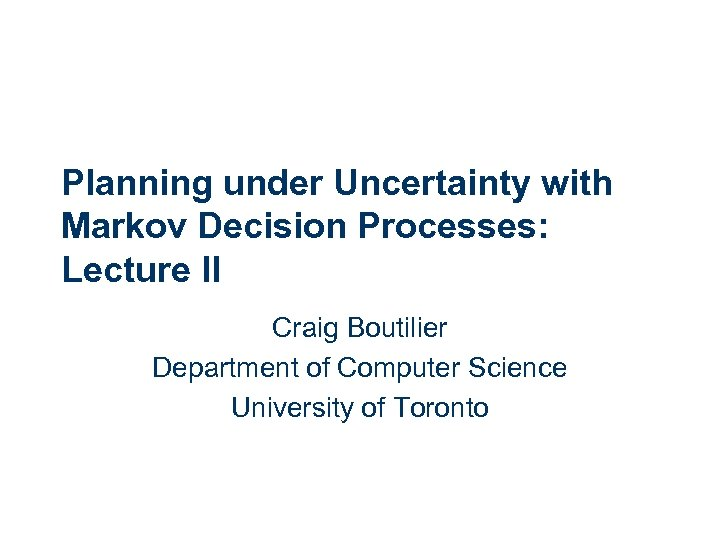Planning under Uncertainty with Markov Decision Processes: Lecture II Craig Boutilier Department of Computer Science University of Toronto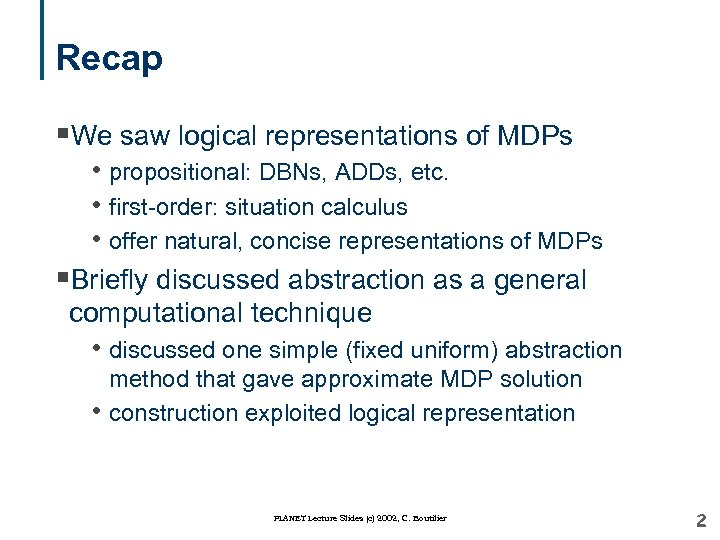Recap §We saw logical representations of MDPs • propositional: DBNs, ADDs, etc. • first-order: situation calculus • offer natural, concise representations of MDPs §Briefly discussed abstraction as a general computational technique • discussed one simple (fixed uniform) abstraction • method that gave approximate MDP solution construction exploited logical representation PLANET Lecture Slides (c) 2002, C. Boutilier 2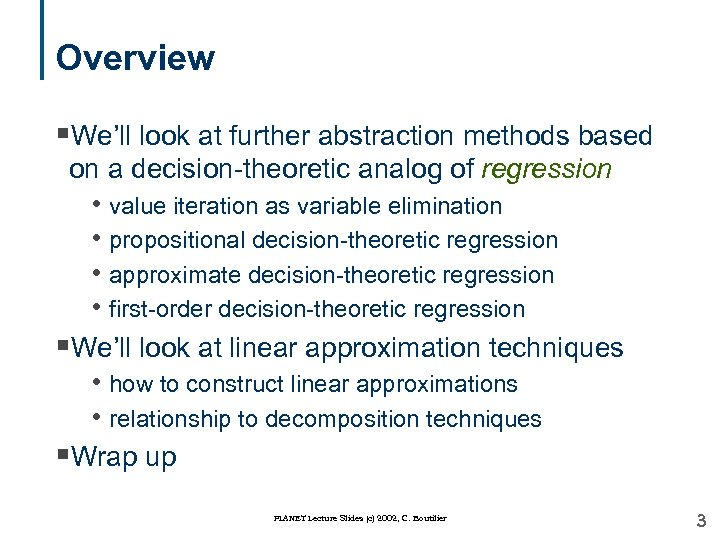Overview §We’ll look at further abstraction methods based on a decision-theoretic analog of regression • value iteration as variable elimination • propositional decision-theoretic regression • approximate decision-theoretic regression • first-order decision-theoretic regression §We’ll look at linear approximation techniques • how to construct linear approximations • relationship to decomposition techniques §Wrap up PLANET Lecture Slides (c) 2002, C. Boutilier 3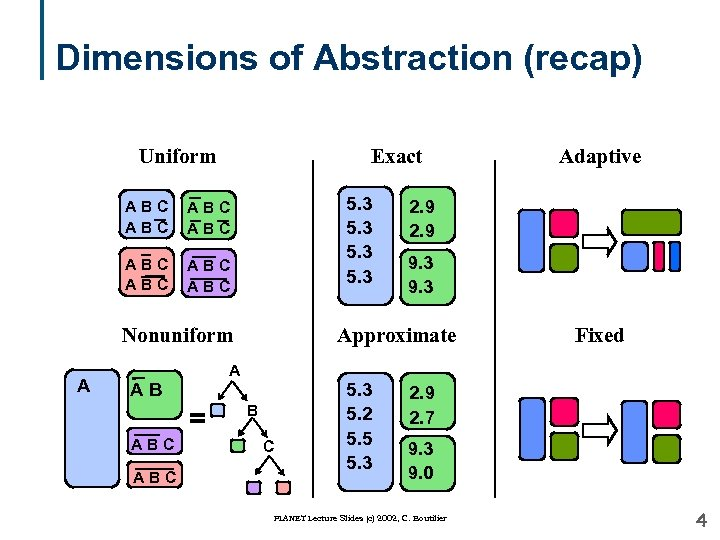Dimensions of Abstraction (recap) Uniform Exact ABC ABC 5. 3 ABC ABC Nonuniform A AB = ABC 2. 9 9. 3 Approximate A B C 5. 3 5. 2 5. 5 5. 3 Adaptive Fixed 2. 9 2. 7 9. 3 9. 0 PLANET Lecture Slides (c) 2002, C. Boutilier 4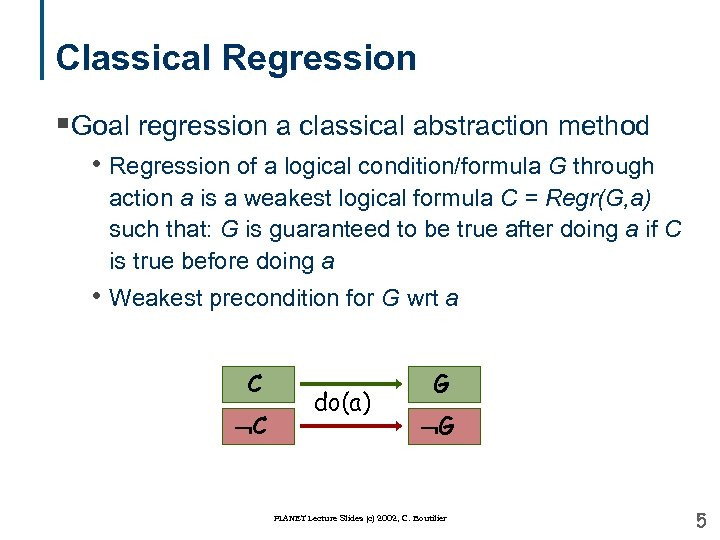Classical Regression §Goal regression a classical abstraction method • Regression of a logical condition/formula G through action a is a weakest logical formula C = Regr(G, a) such that: G is guaranteed to be true after doing a if C is true before doing a • Weakest precondition for G wrt a C C do(a) G G PLANET Lecture Slides (c) 2002, C. Boutilier 5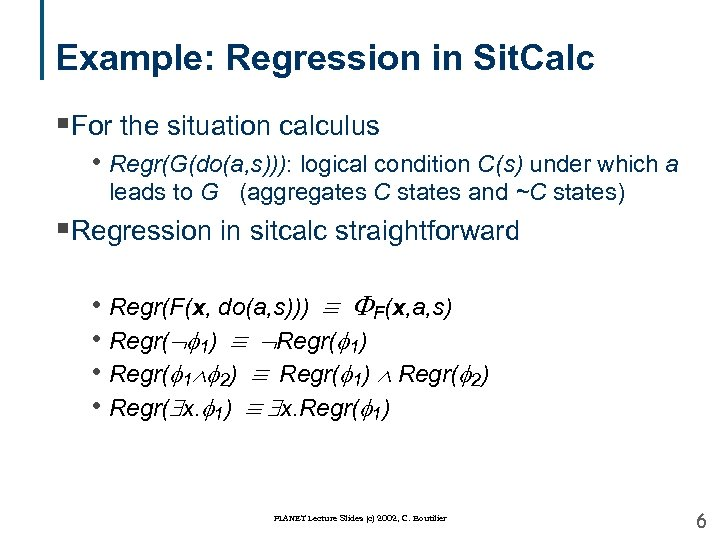Example: Regression in Sit. Calc §For the situation calculus • Regr(G(do(a, s))): logical condition C(s) under which a leads to G (aggregates C states and ~C states) §Regression in sitcalc straightforward • Regr(F(x, do(a, s))) F(x, a, s) • Regr( 1) • Regr( 1 2) Regr( 1) Regr( 2) • Regr( x. 1) x. Regr( 1) PLANET Lecture Slides (c) 2002, C. Boutilier 6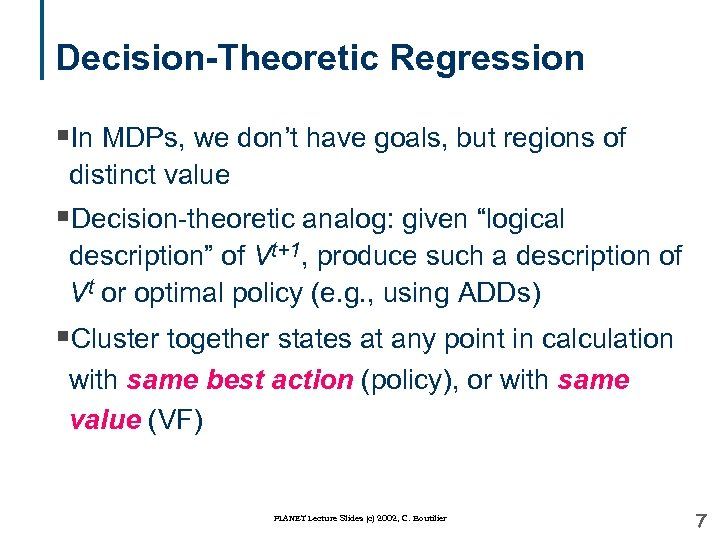Decision-Theoretic Regression §In MDPs, we don’t have goals, but regions of distinct value §Decision-theoretic analog: given “logical description” of Vt+1, produce such a description of Vt or optimal policy (e. g. , using ADDs) §Cluster together states at any point in calculation with same best action (policy), or with same value (VF) PLANET Lecture Slides (c) 2002, C. Boutilier 7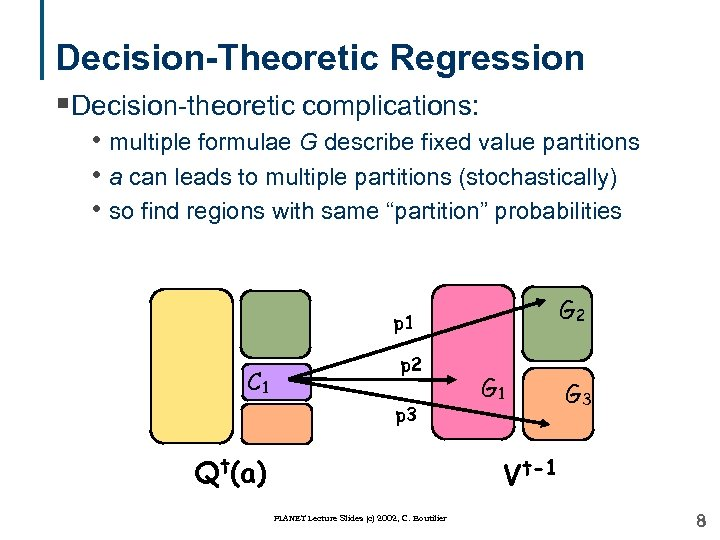Decision-Theoretic Regression §Decision-theoretic complications: • multiple formulae G describe fixed value partitions • a can leads to multiple partitions (stochastically) • so find regions with same “partition” probabilities G 2 p 1 C 1 p 2 p 3 Qt(a) G 1 G 3 Vt-1 PLANET Lecture Slides (c) 2002, C. Boutilier 8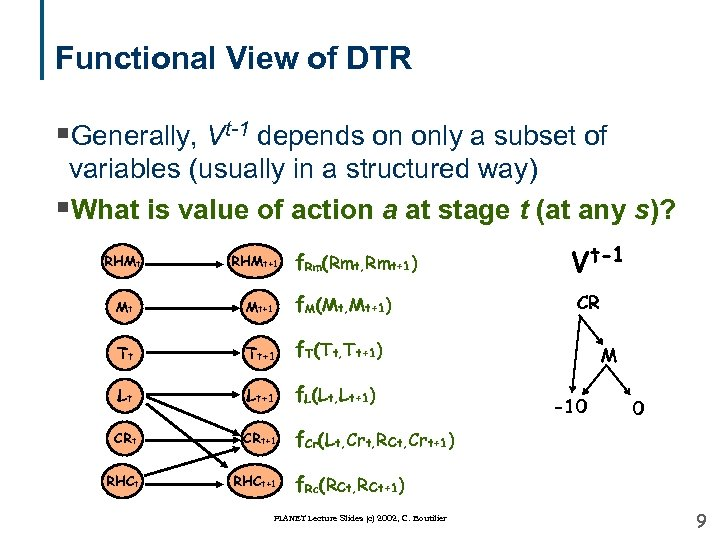Functional View of DTR §Generally, Vt-1 depends on only a subset of variables (usually in a structured way) §What is value of action a at stage t (at any s)? RHMt+1 f. Rm(Rmt, Rmt+1) Mt Mt+1 f. M(Mt, Mt+1) Tt Tt+1 Lt+1 f. L(Lt, Lt+1) CRt+1 f. Cr(Lt, Crt, Rct, Crt+1) RHCt+1 CR f. T(Tt, Tt+1) Lt Vt-1 M -10 0 f. Rc(Rct, Rct+1) PLANET Lecture Slides (c) 2002, C. Boutilier 9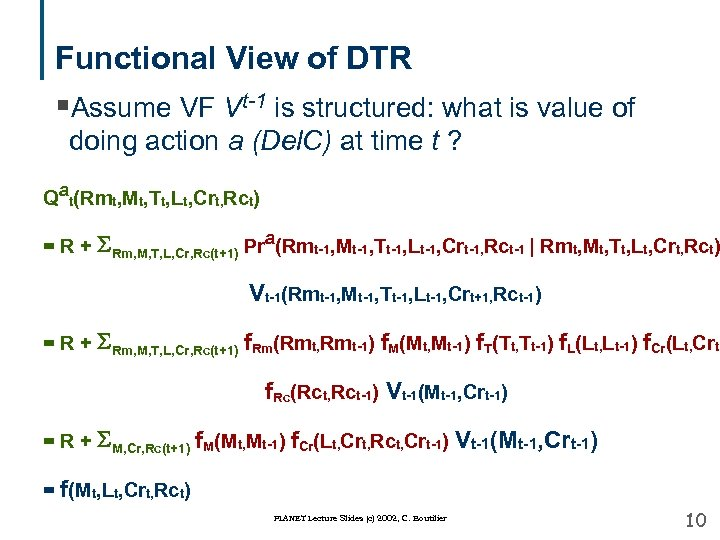Functional View of DTR §Assume VF Vt-1 is structured: what is value of doing action a (Del. C) at time t ? Qat(Rmt, Mt, Tt, Lt, Crt, Rct) = R + SRm, M, T, L, Cr, Rc(t+1) Pra(Rmt-1, Mt-1, Tt-1, Lt-1, Crt-1, Rct-1 | Rmt, Mt, Tt, Lt, Crt, Rct) Vt-1(Rmt-1, Mt-1, Tt-1, Lt-1, Crt+1, Rct-1) = R + SRm, M, T, L, Cr, Rc(t+1) f. Rm(Rmt, Rmt-1) f. M(Mt, Mt-1) f. T(Tt, Tt-1) f. L(Lt, Lt-1) f. Cr(Lt, Crt, f. Rc(Rct, Rct-1) Vt-1(Mt-1, Crt-1) = R + SM, Cr, Rc(t+1) f. M(Mt, Mt-1) f. Cr(Lt, Crt, Rct, Crt-1) Vt-1(Mt-1, Crt-1) = f(Mt, Lt, Crt, Rct) PLANET Lecture Slides (c) 2002, C. Boutilier 10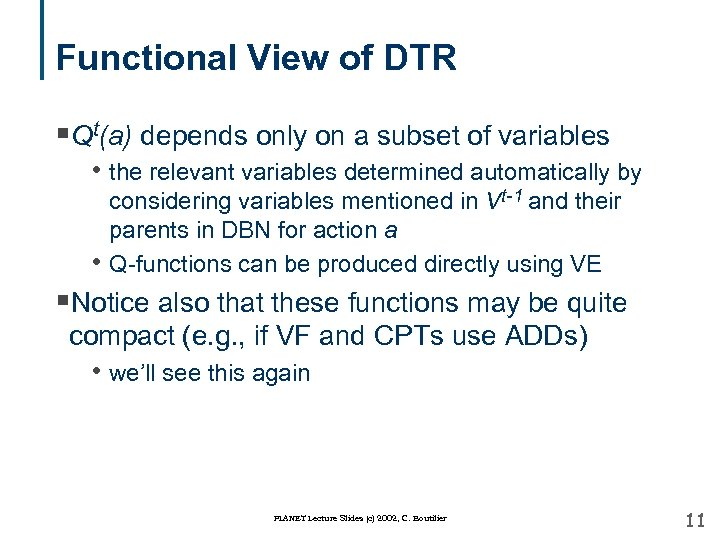Functional View of DTR §Qt(a) depends only on a subset of variables • the relevant variables determined automatically by considering variables mentioned in Vt-1 and their parents in DBN for action a Q-functions can be produced directly using VE • §Notice also that these functions may be quite compact (e. g. , if VF and CPTs use ADDs) • we’ll see this again PLANET Lecture Slides (c) 2002, C. Boutilier 11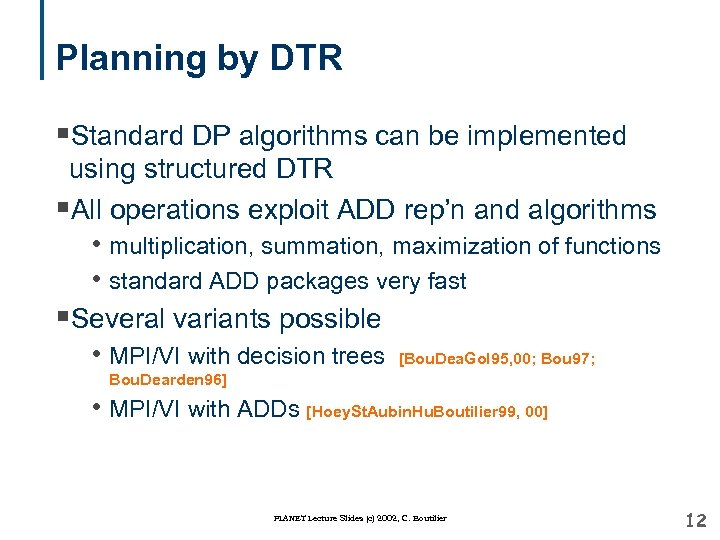Planning by DTR §Standard DP algorithms can be implemented using structured DTR §All operations exploit ADD rep’n and algorithms • multiplication, summation, maximization of functions • standard ADD packages very fast §Several variants possible • MPI/VI with decision trees [Bou. Dea. Gol 95, 00; Bou 97; Bou. Dearden 96] • MPI/VI with ADDs [Hoey. St. Aubin. Hu. Boutilier 99, 00] PLANET Lecture Slides (c) 2002, C. Boutilier 12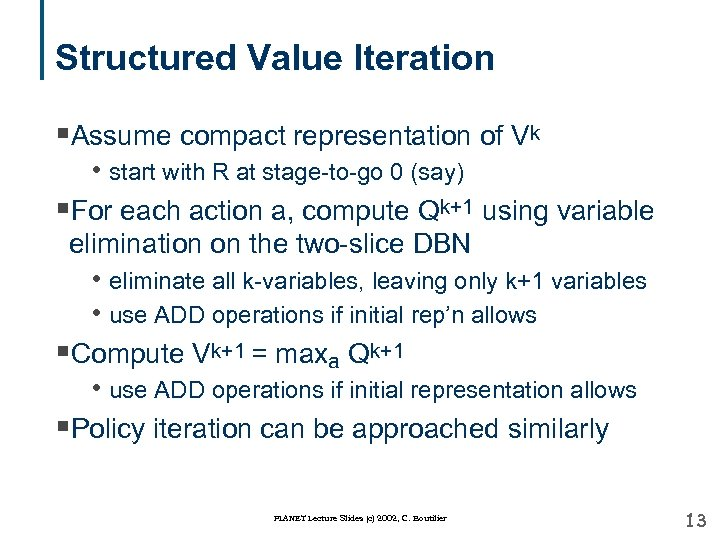Structured Value Iteration §Assume compact representation of Vk • start with R at stage-to-go 0 (say) §For each action a, compute Qk+1 using variable elimination on the two-slice DBN • eliminate all k-variables, leaving only k+1 variables • use ADD operations if initial rep’n allows §Compute Vk+1 = maxa Qk+1 • use ADD operations if initial representation allows §Policy iteration can be approached similarly PLANET Lecture Slides (c) 2002, C. Boutilier 13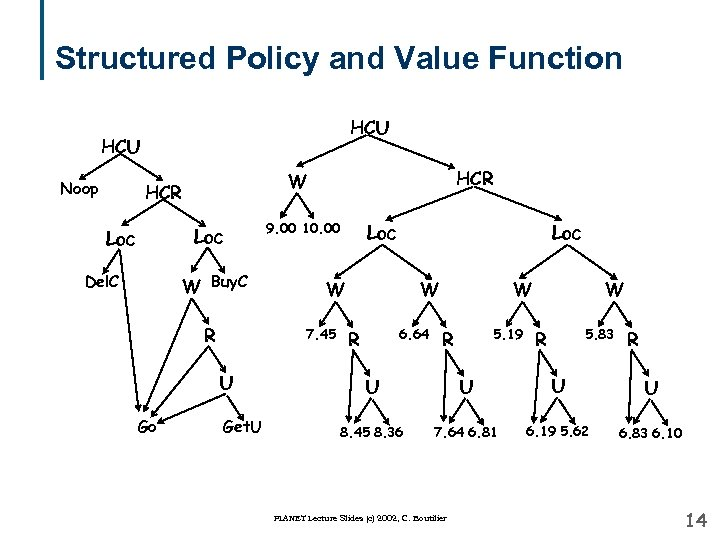Structured Policy and Value Function HCU Noop Loc Del. C W Buy. C R 9. 00 10. 00 Get. U Loc W 7. 45 U Go HCR W 6. 64 R W 5. 19 R W 5. 83 R R U U 8. 45 8. 36 7. 64 6. 81 6. 19 5. 62 6. 83 6. 10 PLANET Lecture Slides (c) 2002, C. Boutilier 14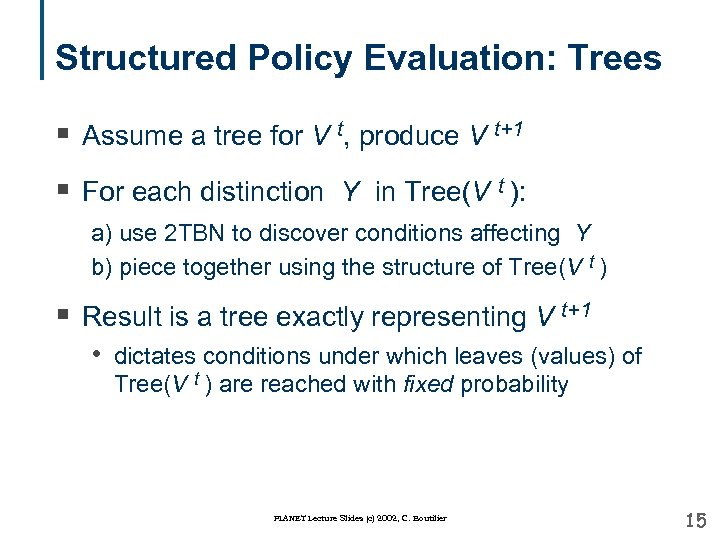Structured Policy Evaluation: Trees § Assume a tree for V t, produce V t+1 § For each distinction Y in Tree(V t ): a) use 2 TBN to discover conditions affecting Y b) piece together using the structure of Tree(V t ) § Result is a tree exactly representing V t+1 • dictates conditions under which leaves (values) of Tree(V t ) are reached with fixed probability PLANET Lecture Slides (c) 2002, C. Boutilier 15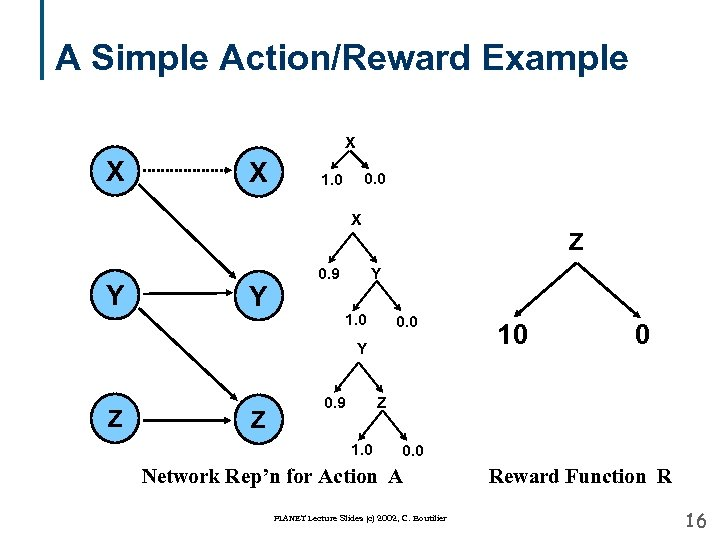A Simple Action/Reward Example X X X 0. 0 1. 0 X Z Y Y 0. 9 Y 1. 0 0. 0 Y Z Z 0. 9 10 0 Z 1. 0 0. 0 Network Rep’n for Action A PLANET Lecture Slides (c) 2002, C. Boutilier Reward Function R 16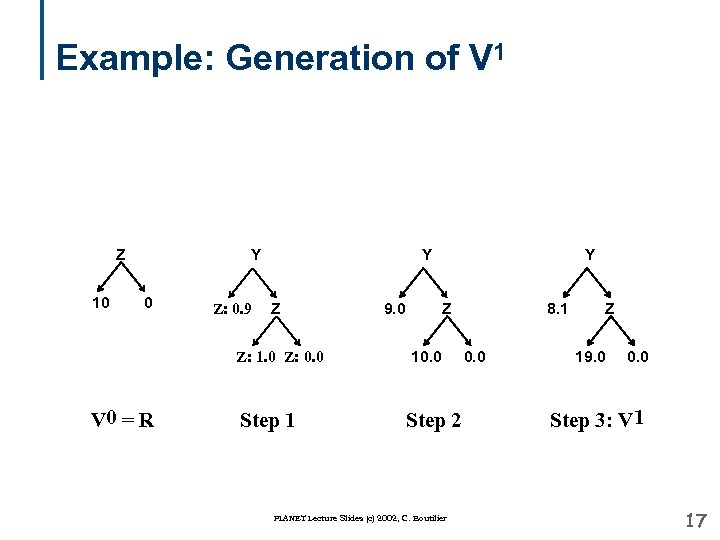Example: Generation of V 1 Y Z 10 0 Z: 0. 9 Y Z Z: 1. 0 Z: 0. 0 V 0 = R Step 1 9. 0 Y Z 10. 0 Step 2 PLANET Lecture Slides (c) 2002, C. Boutilier 8. 1 0. 0 Z 19. 0 0. 0 Step 3: V 1 17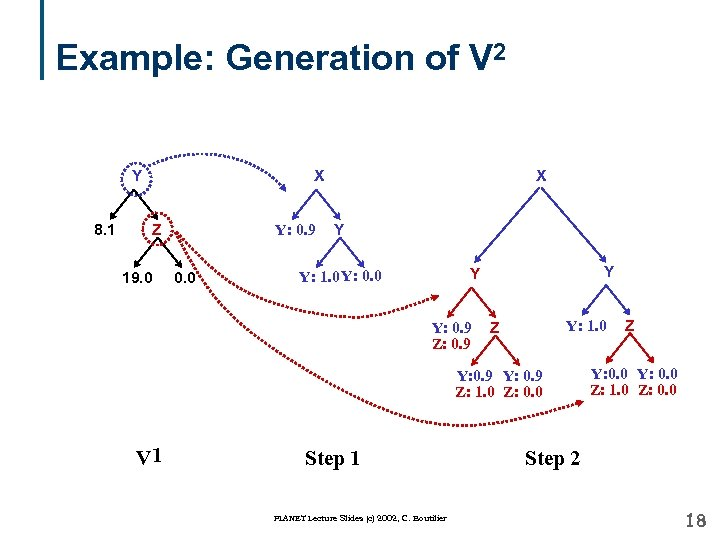Example: Generation of V 2 Y 8. 1 X Z 19. 0 Y: 0. 9 0. 0 X Y Y: 1. 0 Y: 0. 9 Z: 0. 9 Y: 1. 0 Z Y: 0. 9 Z: 1. 0 Z: 0. 0 V 1 Step 1 PLANET Lecture Slides (c) 2002, C. Boutilier Z Y: 0. 0 Z: 1. 0 Z: 0. 0 Step 2 18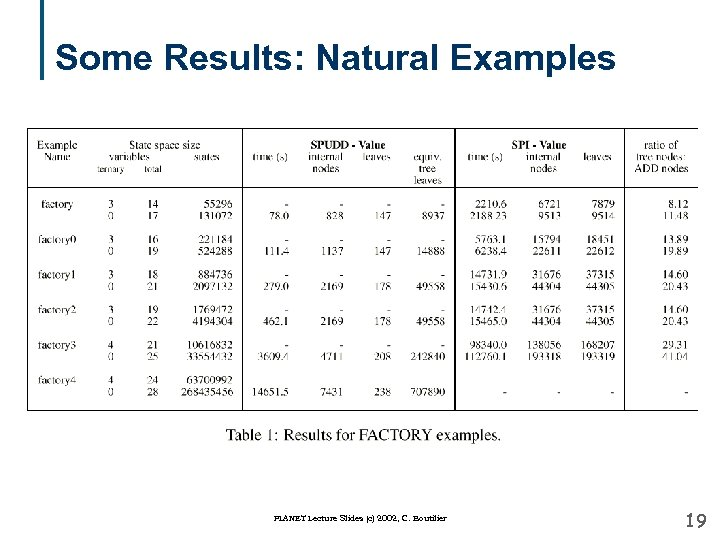Some Results: Natural Examples PLANET Lecture Slides (c) 2002, C. Boutilier 19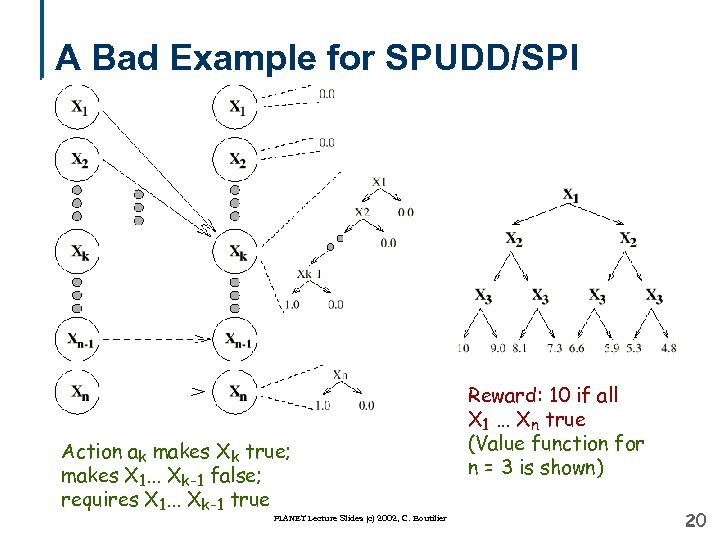A Bad Example for SPUDD/SPI Action ak makes Xk true; makes X 1. . . Xk-1 false; requires X 1. . . Xk-1 true PLANET Lecture Slides (c) 2002, C. Boutilier Reward: 10 if all X 1. . . Xn true (Value function for n = 3 is shown) 20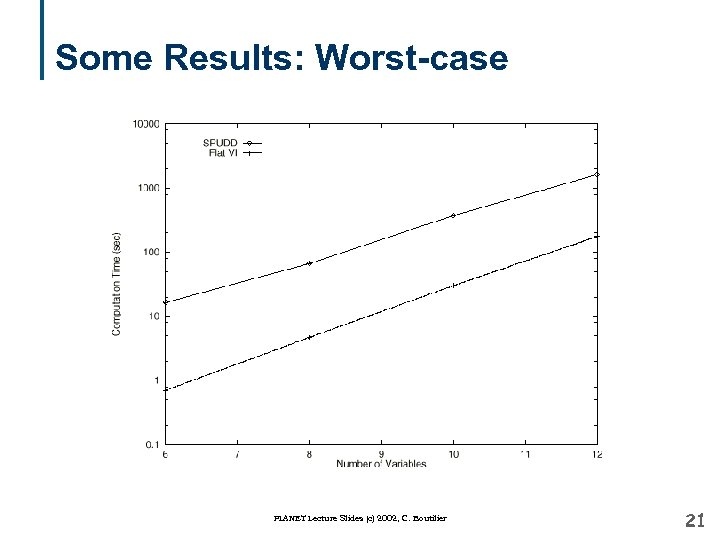Some Results: Worst-case PLANET Lecture Slides (c) 2002, C. Boutilier 21A Good Example for SPUDD/SPI Action ak makes Xk true; requires X 1. . . Xk-1 true PLANET Lecture Slides (c) 2002, C. Boutilier Reward: 10 if all X 1. . . Xn true (Value function for n = 3 is shown) 22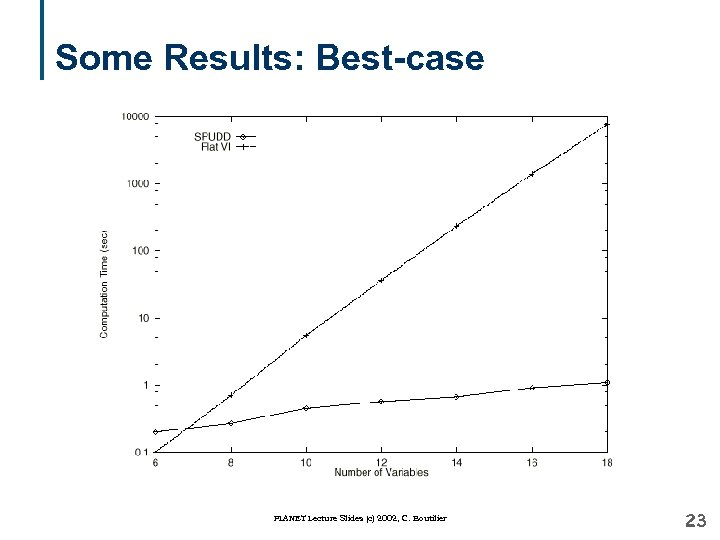Some Results: Best-case PLANET Lecture Slides (c) 2002, C. Boutilier 23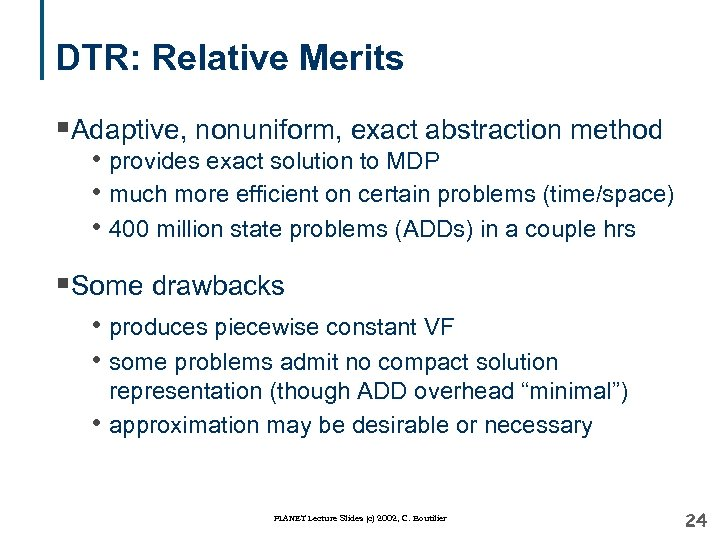DTR: Relative Merits §Adaptive, nonuniform, exact abstraction method • provides exact solution to MDP • much more efficient on certain problems (time/space) • 400 million state problems (ADDs) in a couple hrs §Some drawbacks • produces piecewise constant VF • some problems admit no compact solution • representation (though ADD overhead “minimal”) approximation may be desirable or necessary PLANET Lecture Slides (c) 2002, C. Boutilier 24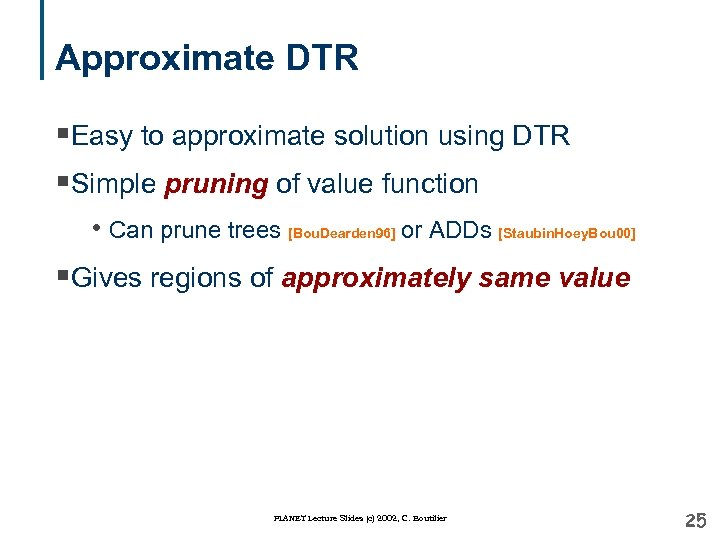Approximate DTR §Easy to approximate solution using DTR §Simple pruning of value function • Can prune trees [Bou. Dearden 96] or ADDs [Staubin. Hoey. Bou 00] §Gives regions of approximately same value PLANET Lecture Slides (c) 2002, C. Boutilier 25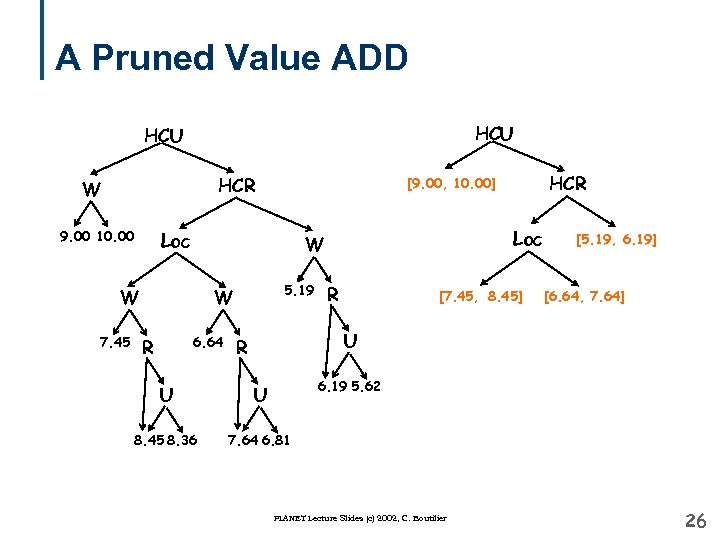A Pruned Value ADD HCU HCR W 9. 00 10. 00 Loc 7. 45 5. 19 W 6. 64 R Loc W W HCR [9. 00, 10. 00] R [7. 45, 8. 45] [5. 19, 6. 19] [6. 64, 7. 64] U R 6. 19 5. 62 U U 8. 45 8. 36 7. 64 6. 81 PLANET Lecture Slides (c) 2002, C. Boutilier 26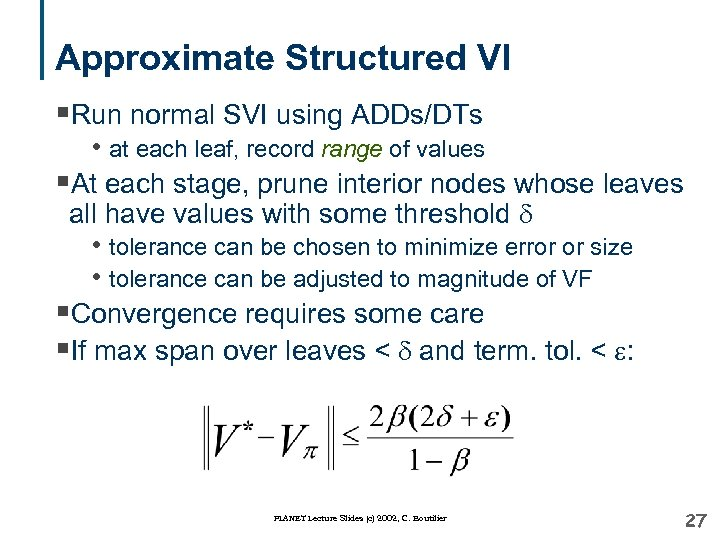Approximate Structured VI §Run normal SVI using ADDs/DTs • at each leaf, record range of values §At each stage, prune interior nodes whose leaves all have values with some threshold d • tolerance can be chosen to minimize error or size • tolerance can be adjusted to magnitude of VF §Convergence requires some care §If max span over leaves < d and term. tol. < e: PLANET Lecture Slides (c) 2002, C. Boutilier 27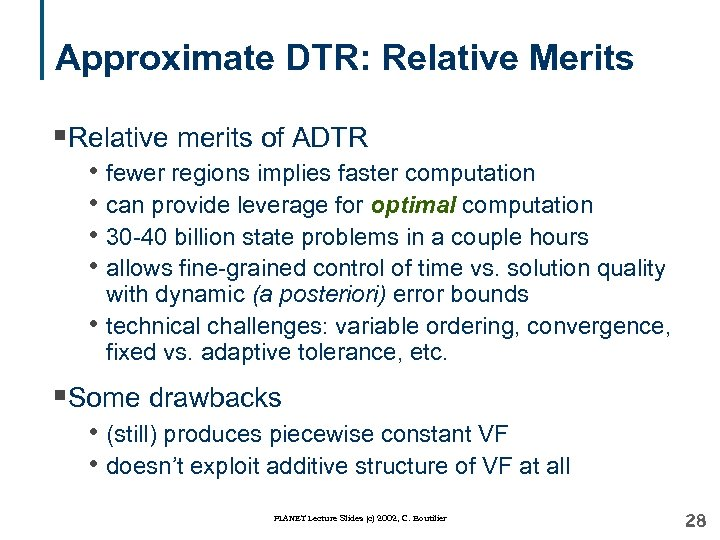Approximate DTR: Relative Merits §Relative merits of ADTR • fewer regions implies faster computation • can provide leverage for optimal computation • 30 -40 billion state problems in a couple hours • allows fine-grained control of time vs. solution quality • with dynamic (a posteriori) error bounds technical challenges: variable ordering, convergence, fixed vs. adaptive tolerance, etc. §Some drawbacks • (still) produces piecewise constant VF • doesn’t exploit additive structure of VF at all PLANET Lecture Slides (c) 2002, C. Boutilier 28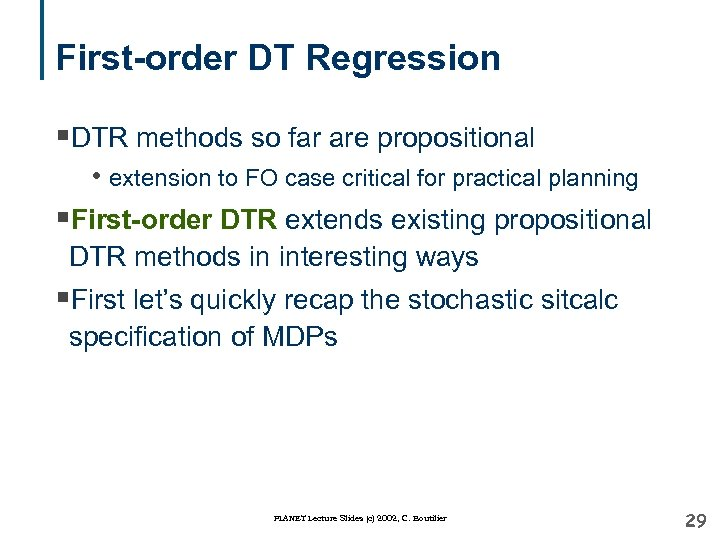First-order DT Regression §DTR methods so far are propositional • extension to FO case critical for practical planning §First-order DTR extends existing propositional DTR methods in interesting ways §First let’s quickly recap the stochastic sitcalc specification of MDPs PLANET Lecture Slides (c) 2002, C. Boutilier 29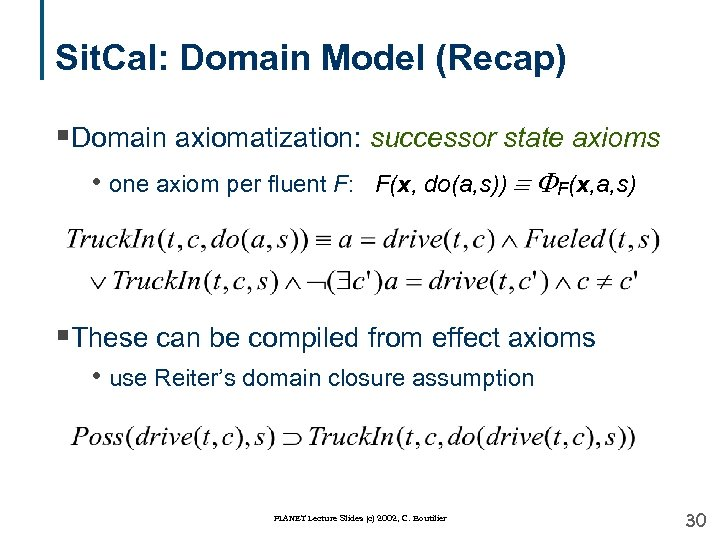Sit. Cal: Domain Model (Recap) §Domain axiomatization: successor state axioms • one axiom per fluent F: F(x, do(a, s)) F(x, a, s) §These can be compiled from effect axioms • use Reiter’s domain closure assumption PLANET Lecture Slides (c) 2002, C. Boutilier 30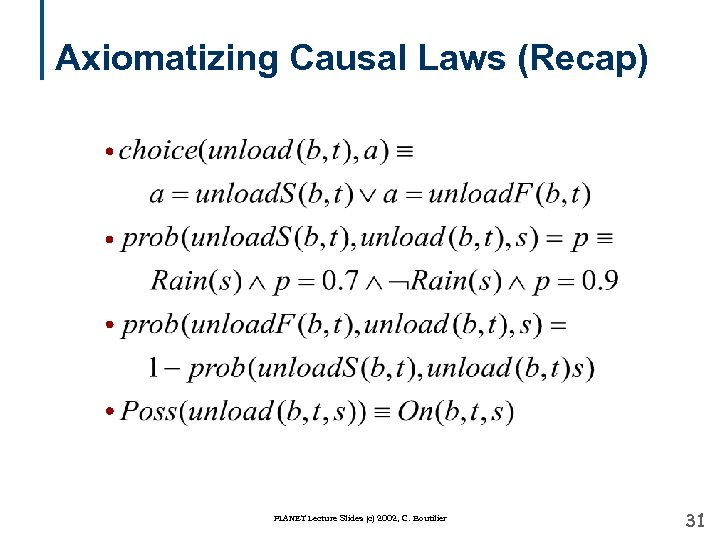Axiomatizing Causal Laws (Recap) PLANET Lecture Slides (c) 2002, C. Boutilier 31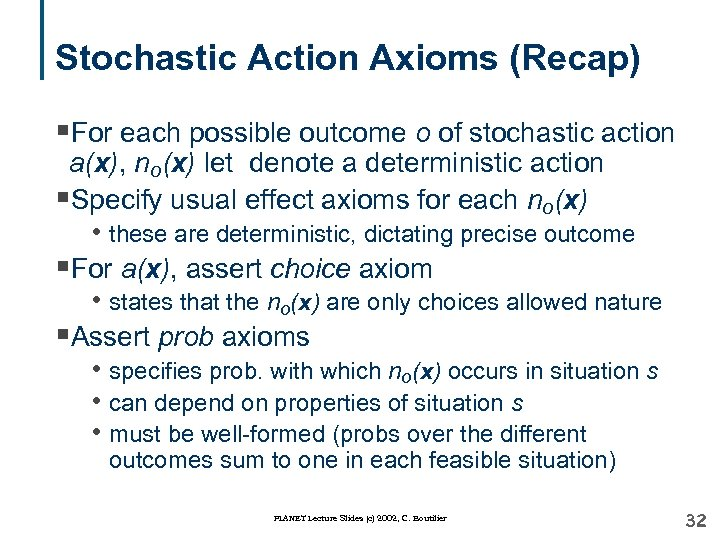Stochastic Action Axioms (Recap) §For each possible outcome o of stochastic action a(x), no(x) let denote a deterministic action §Specify usual effect axioms for each no(x) • these are deterministic, dictating precise outcome §For a(x), assert choice axiom • states that the no(x) are only choices allowed nature §Assert prob axioms • specifies prob. with which no(x) occurs in situation s • can depend on properties of situation s • must be well-formed (probs over the different outcomes sum to one in each feasible situation) PLANET Lecture Slides (c) 2002, C. Boutilier 32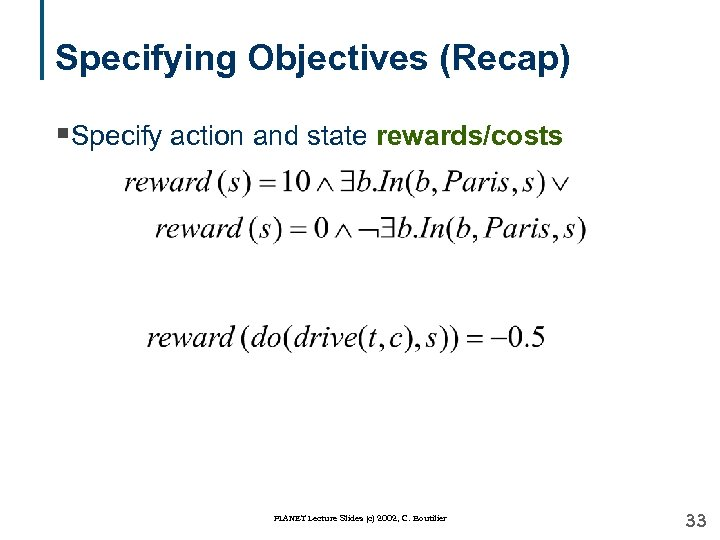Specifying Objectives (Recap) §Specify action and state rewards/costs PLANET Lecture Slides (c) 2002, C. Boutilier 33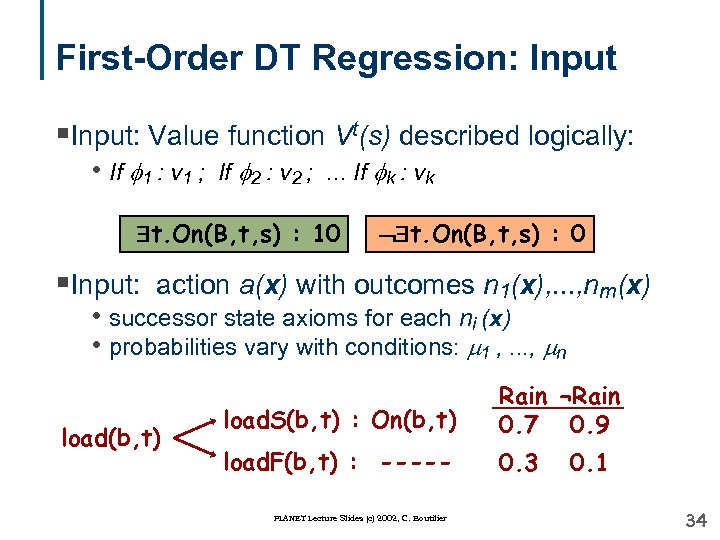First-Order DT Regression: Input §Input: Value function Vt(s) described logically: • If 1 : v 1 ; If 2 : v 2 ; . . . If k : vk t. On(B, t, s) : 10 t. On(B, t, s) : 0 §Input: action a(x) with outcomes n 1(x), . . . , nm(x) • successor state axioms for each ni (x) • probabilities vary with conditions: 1 , . . . , n load(b, t) load. S(b, t) : On(b, t) Rain ¬Rain 0. 7 0. 9 load. F(b, t) : ----- 0. 3 PLANET Lecture Slides (c) 2002, C. Boutilier 0. 1 34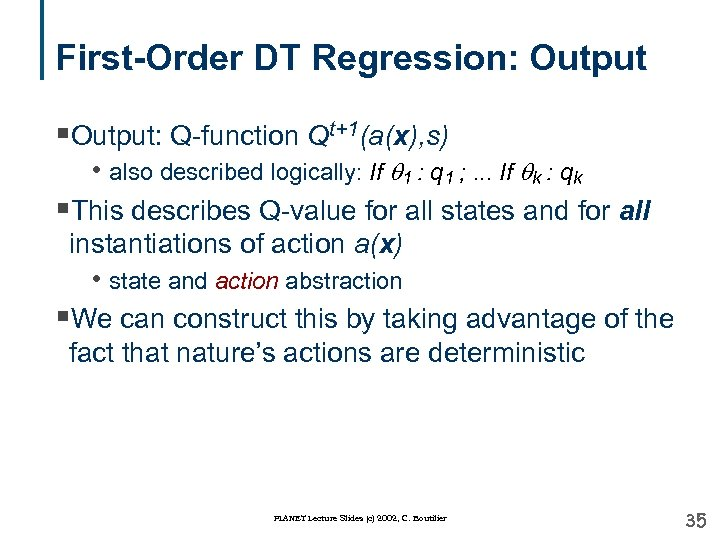First-Order DT Regression: Output §Output: Q-function Qt+1(a(x), s) • also described logically: If q 1 : q 1 ; . . . If qk : qk §This describes Q-value for all states and for all instantiations of action a(x) • state and action abstraction §We can construct this by taking advantage of the fact that nature’s actions are deterministic PLANET Lecture Slides (c) 2002, C. Boutilier 35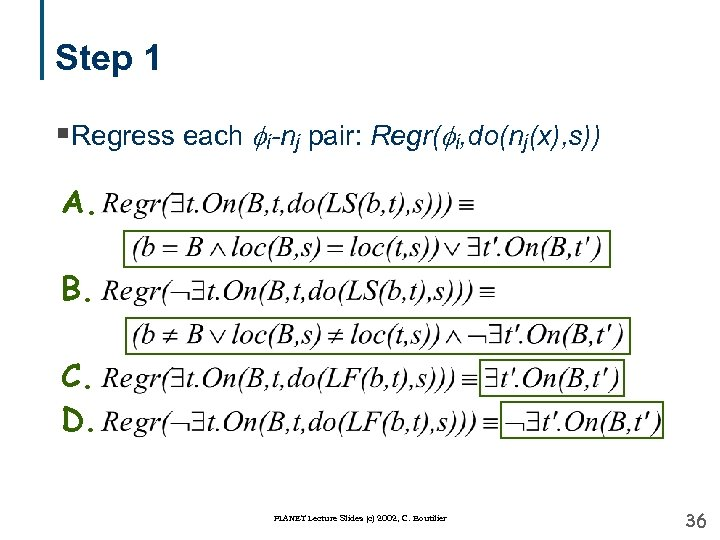Step 1 §Regress each i-nj pair: Regr( i, do(nj(x), s)) A. B. C. D. PLANET Lecture Slides (c) 2002, C. Boutilier 36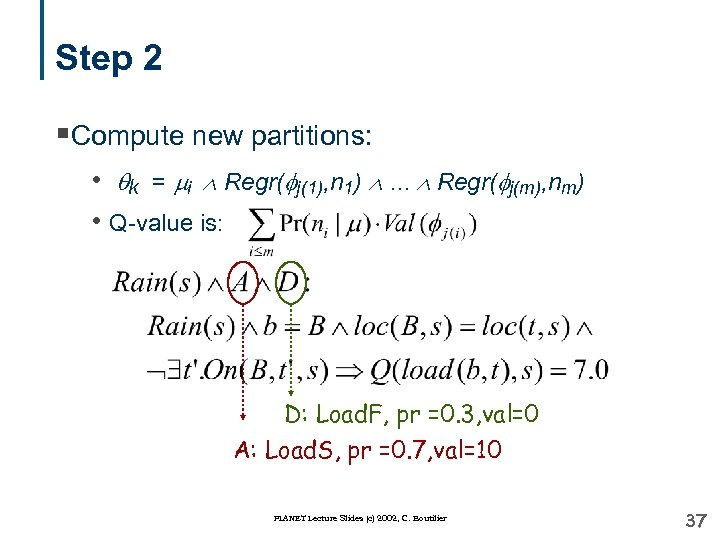Step 2 §Compute new partitions: • qk = i Regr( j(1), n 1) . . . Regr( j(m), nm) • Q-value is: D: Load. F, pr =0. 3, val=0 A: Load. S, pr =0. 7, val=10 PLANET Lecture Slides (c) 2002, C. Boutilier 37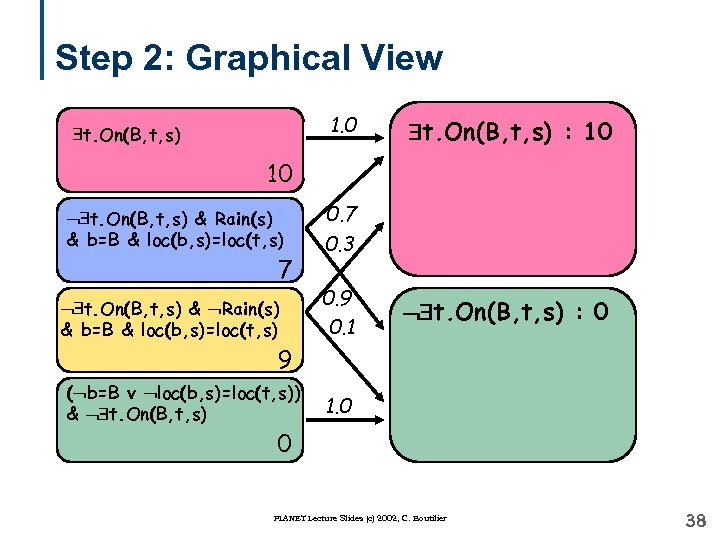Step 2: Graphical View 1. 0 t. On(B, t, s) : 10 10 t. On(B, t, s) & Rain(s) & b=B & loc(b, s)=loc(t, s) 7 t. On(B, t, s) & Rain(s) & b=B & loc(b, s)=loc(t, s) 0. 7 0. 3 0. 9 0. 1 t. On(B, t, s) : 0 9 ( b=B v loc(b, s)=loc(t, s)) & t. On(B, t, s) 1. 0 0 PLANET Lecture Slides (c) 2002, C. Boutilier 38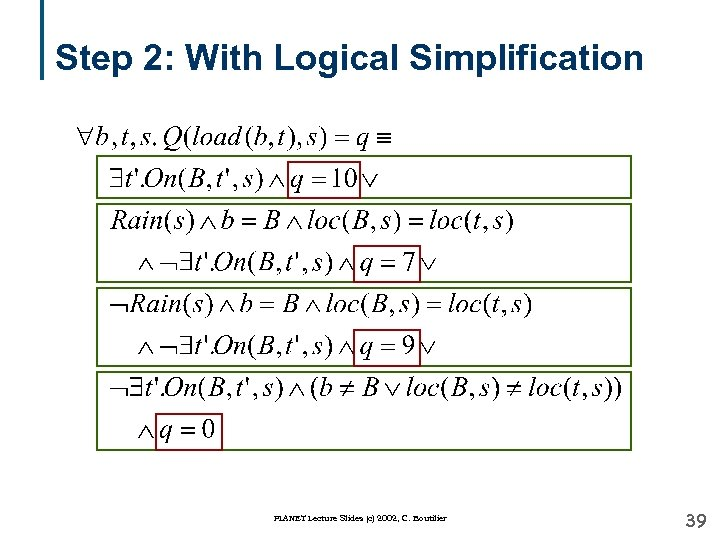Step 2: With Logical Simplification PLANET Lecture Slides (c) 2002, C. Boutilier 39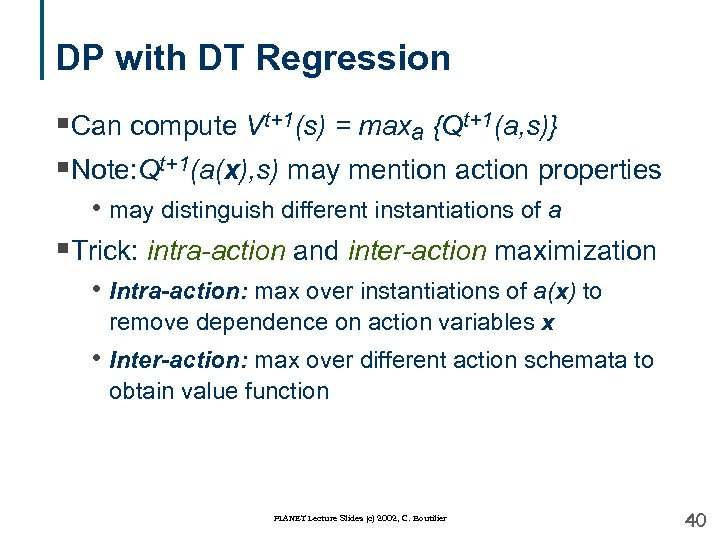DP with DT Regression §Can compute Vt+1(s) = maxa {Qt+1(a, s)} §Note: Qt+1(a(x), s) may mention action properties • may distinguish different instantiations of a §Trick: intra-action and inter-action maximization • Intra-action: max over instantiations of a(x) to remove dependence on action variables x • Inter-action: max over different action schemata to obtain value function PLANET Lecture Slides (c) 2002, C. Boutilier 40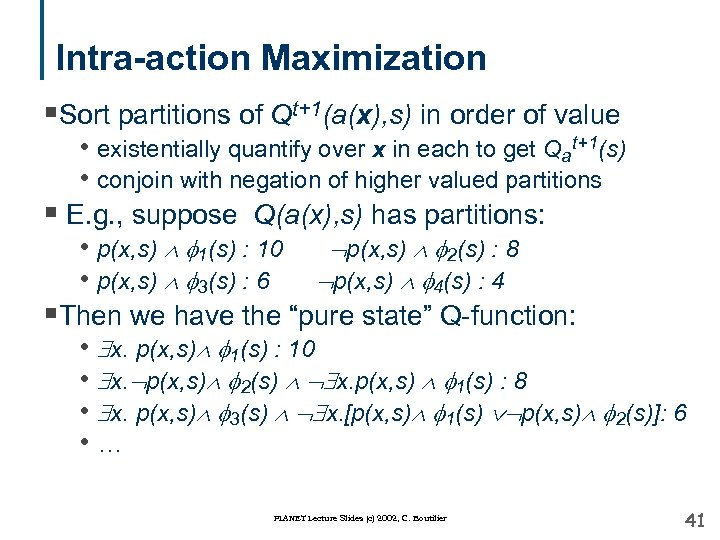Intra-action Maximization §Sort partitions of Qt+1(a(x), s) in order of value • existentially quantify over x in each to get Qat+1(s) • conjoin with negation of higher valued partitions § E. g. , suppose Q(a(x), s) has partitions: • p(x, s) 1(s) : 10 p(x, s) 2(s) : 8 • p(x, s) 3(s) : 6 p(x, s) 4(s) : 4 §Then we have the “pure state” Q-function: • x. p(x, s) 1(s) : 10 • x. p(x, s) 2(s) x. p(x, s) 1(s) : 8 • x. p(x, s) 3(s) x. [p(x, s) 1(s) p(x, s) 2(s)]: 6 • … PLANET Lecture Slides (c) 2002, C. Boutilier 41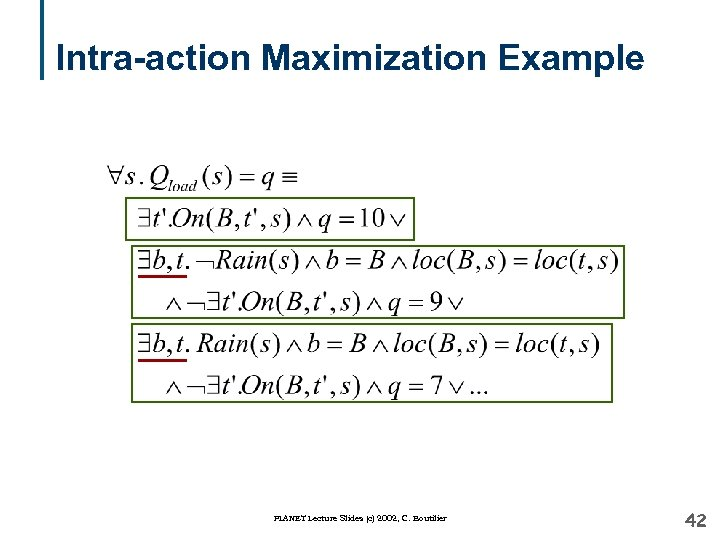Intra-action Maximization Example PLANET Lecture Slides (c) 2002, C. Boutilier 42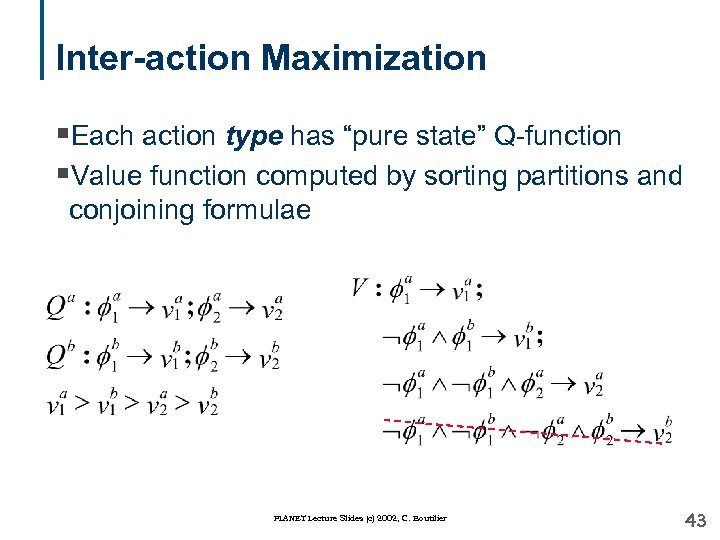Inter-action Maximization §Each action type has “pure state” Q-function §Value function computed by sorting partitions and conjoining formulae PLANET Lecture Slides (c) 2002, C. Boutilier 43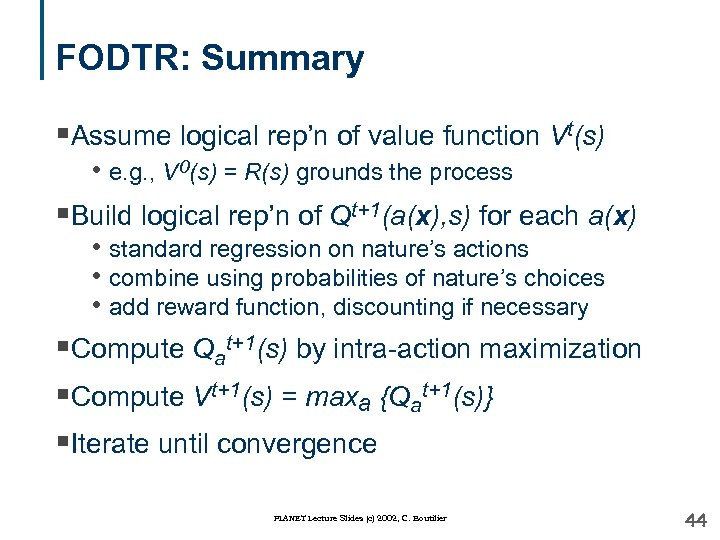FODTR: Summary §Assume logical rep’n of value function Vt(s) • e. g. , V 0(s) = R(s) grounds the process §Build logical rep’n of Qt+1(a(x), s) for each a(x) • standard regression on nature’s actions • combine using probabilities of nature’s choices • add reward function, discounting if necessary §Compute Qat+1(s) by intra-action maximization §Compute Vt+1(s) = maxa {Qat+1(s)} §Iterate until convergence PLANET Lecture Slides (c) 2002, C. Boutilier 44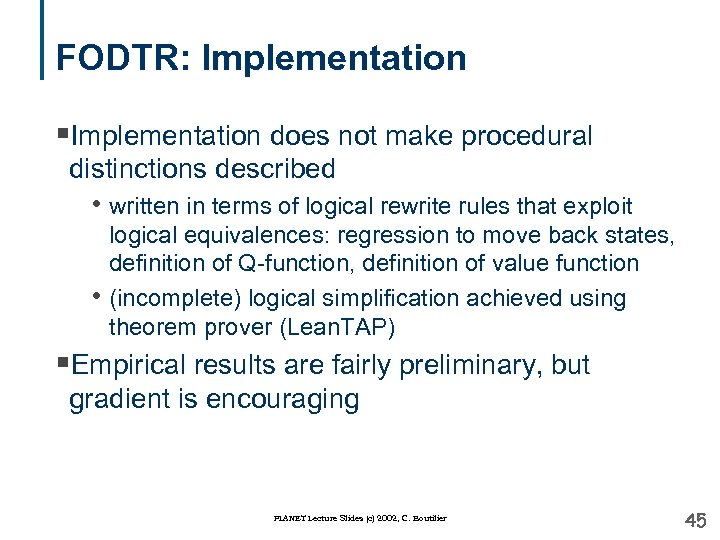FODTR: Implementation §Implementation does not make procedural distinctions described • written in terms of logical rewrite rules that exploit • logical equivalences: regression to move back states, definition of Q-function, definition of value function (incomplete) logical simplification achieved using theorem prover (Lean. TAP) §Empirical results are fairly preliminary, but gradient is encouraging PLANET Lecture Slides (c) 2002, C. Boutilier 45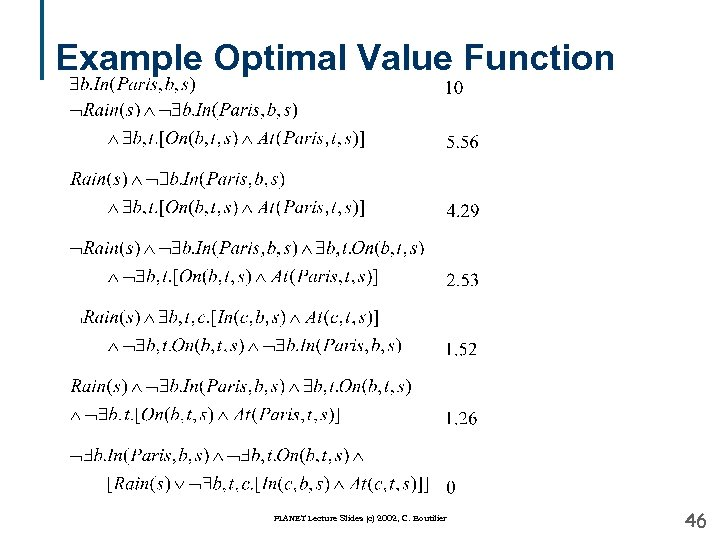Example Optimal Value Function PLANET Lecture Slides (c) 2002, C. Boutilier 46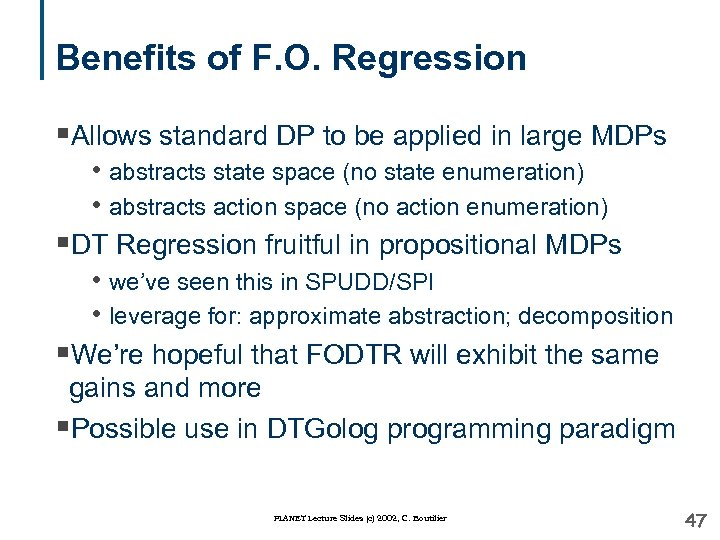Benefits of F. O. Regression §Allows standard DP to be applied in large MDPs • abstracts state space (no state enumeration) • abstracts action space (no action enumeration) §DT Regression fruitful in propositional MDPs • we’ve seen this in SPUDD/SPI • leverage for: approximate abstraction; decomposition §We’re hopeful that FODTR will exhibit the same gains and more §Possible use in DTGolog programming paradigm PLANET Lecture Slides (c) 2002, C. Boutilier 47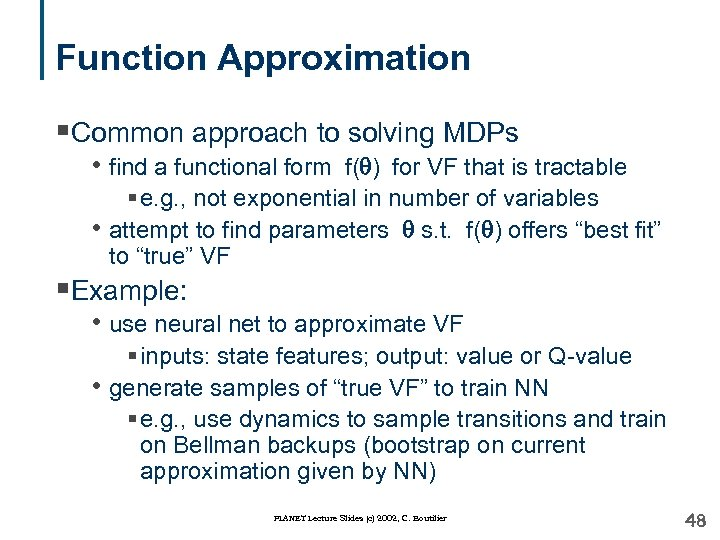Function Approximation §Common approach to solving MDPs • find a functional form f(q) for VF that is tractable § e. g. , not exponential in number of variables • attempt to find parameters q s. t. f(q) offers “best fit” to “true” VF §Example: • use neural net to approximate VF § inputs: state features; output: value or Q-value • generate samples of “true VF” to train NN § e. g. , use dynamics to sample transitions and train on Bellman backups (bootstrap on current approximation given by NN) PLANET Lecture Slides (c) 2002, C. Boutilier 48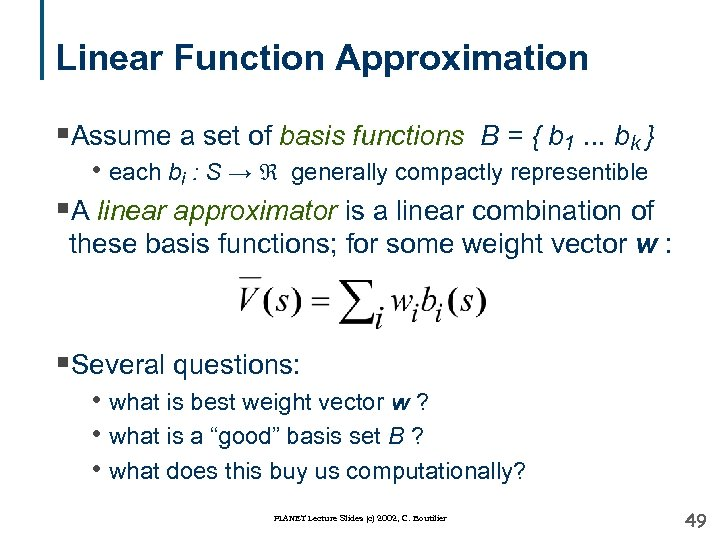Linear Function Approximation §Assume a set of basis functions B = { b 1. . . bk } • each bi : S → generally compactly representible §A linear approximator is a linear combination of these basis functions; for some weight vector w : §Several questions: • what is best weight vector w ? • what is a “good” basis set B ? • what does this buy us computationally? PLANET Lecture Slides (c) 2002, C. Boutilier 49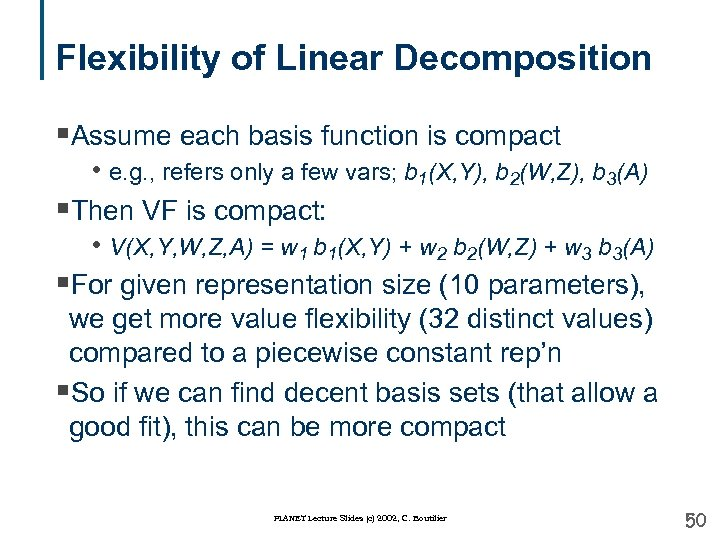Flexibility of Linear Decomposition §Assume each basis function is compact • e. g. , refers only a few vars; b 1(X, Y), b 2(W, Z), b 3(A) §Then VF is compact: • V(X, Y, W, Z, A) = w 1 b 1(X, Y) + w 2 b 2(W, Z) + w 3 b 3(A) §For given representation size (10 parameters), we get more value flexibility (32 distinct values) compared to a piecewise constant rep’n §So if we can find decent basis sets (that allow a good fit), this can be more compact PLANET Lecture Slides (c) 2002, C. Boutilier 50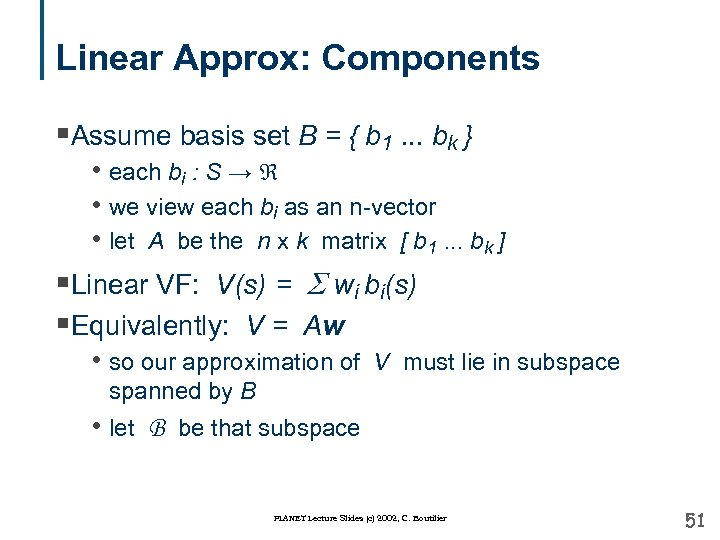Linear Approx: Components §Assume basis set B = { b 1. . . bk } • each bi : S → • we view each bi as an n-vector • let A be the n x k matrix [ b 1. . . bk ] §Linear VF: V(s) = S wi bi(s) §Equivalently: V = Aw • so our approximation of V must lie in subspace spanned by B • let B be that subspace PLANET Lecture Slides (c) 2002, C. Boutilier 51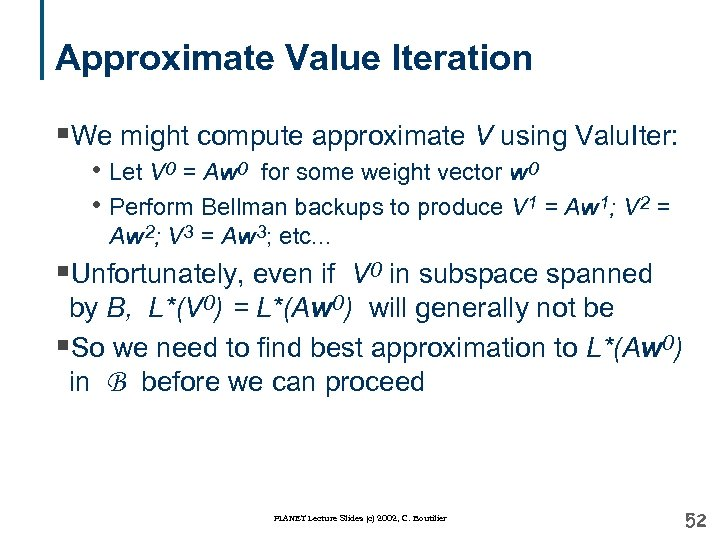Approximate Value Iteration §We might compute approximate V using Valu. Iter: • Let V 0 = Aw 0 for some weight vector w 0 • Perform Bellman backups to produce V 1 = Aw 1; V 2 = Aw 2; V 3 = Aw 3; etc. . . §Unfortunately, even if V 0 in subspace spanned by B, L*(V 0) = L*(Aw 0) will generally not be §So we need to find best approximation to L*(Aw 0) in B before we can proceed PLANET Lecture Slides (c) 2002, C. Boutilier 52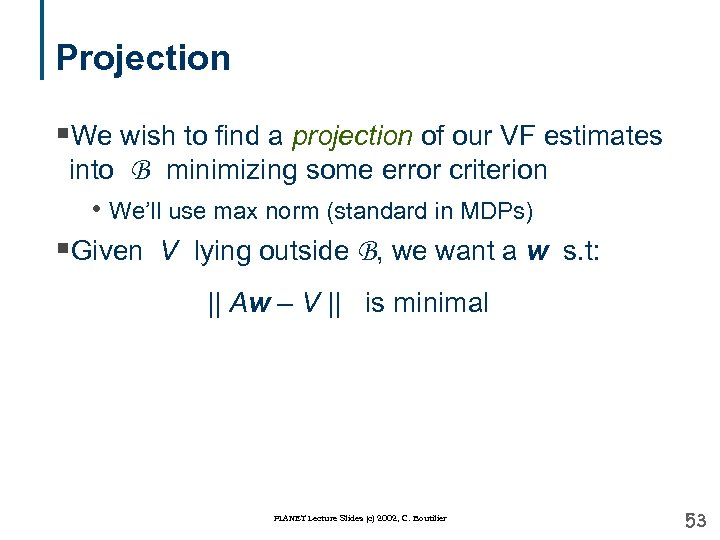Projection §We wish to find a projection of our VF estimates into B minimizing some error criterion • We’ll use max norm (standard in MDPs) §Given V lying outside B, we want a w s. t: || Aw – V || is minimal PLANET Lecture Slides (c) 2002, C. Boutilier 53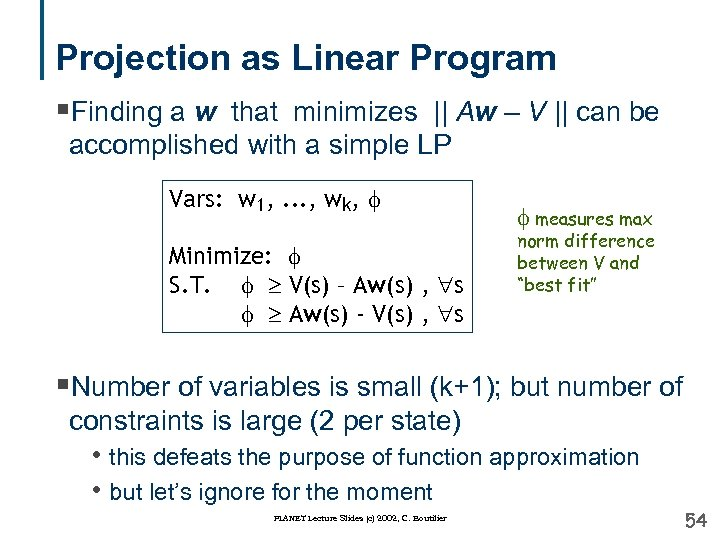Projection as Linear Program §Finding a w that minimizes || Aw – V || can be accomplished with a simple LP Vars: w 1, . . . , wk, Minimize: S. T. V(s) – Aw(s) , s Aw(s) - V(s) , s measures max norm difference between V and “best fit” §Number of variables is small (k+1); but number of constraints is large (2 per state) • this defeats the purpose of function approximation • but let’s ignore for the moment PLANET Lecture Slides (c) 2002, C. Boutilier 54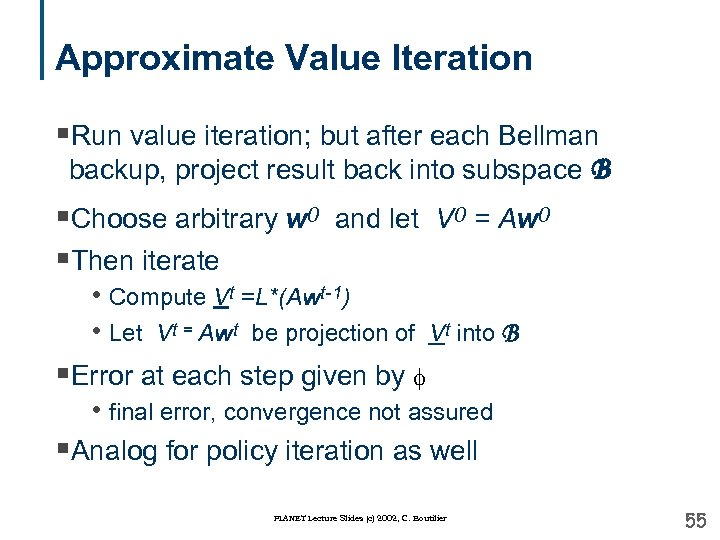Approximate Value Iteration §Run value iteration; but after each Bellman backup, project result back into subspace B §Choose arbitrary w 0 and let V 0 = Aw 0 §Then iterate • Compute Vt =L*(Awt-1) • Let Vt = Awt be projection of Vt into B §Error at each step given by • final error, convergence not assured §Analog for policy iteration as well PLANET Lecture Slides (c) 2002, C. Boutilier 55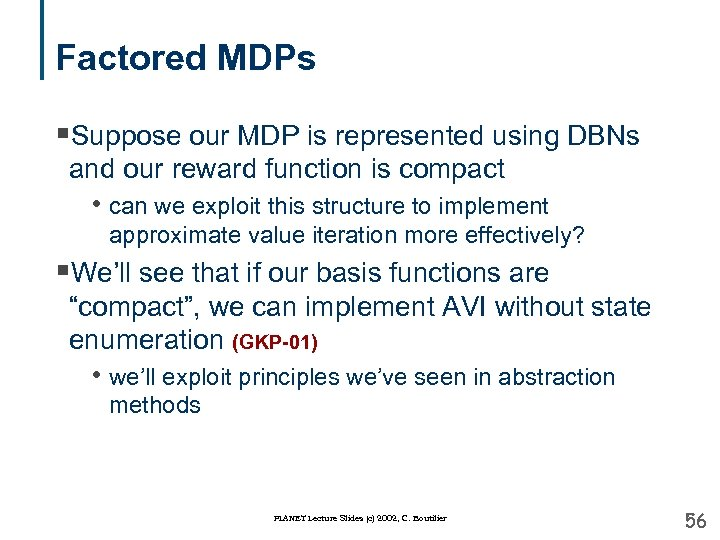Factored MDPs §Suppose our MDP is represented using DBNs and our reward function is compact • can we exploit this structure to implement approximate value iteration more effectively? §We’ll see that if our basis functions are “compact”, we can implement AVI without state enumeration (GKP-01) • we’ll exploit principles we’ve seen in abstraction methods PLANET Lecture Slides (c) 2002, C. Boutilier 56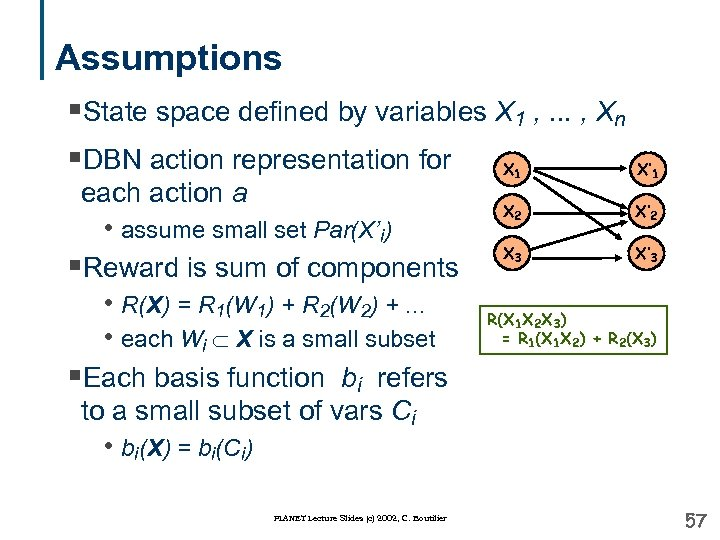Assumptions §State space defined by variables X 1 , . . . , Xn §DBN action representation for X each action a • assume small set Par(X’i) §Reward is sum of components • R(X) = R 1(W 1) + R 2(W 2) +. . . • each Wi X is a small subset §Each basis function bi refers to a small subset of vars Ci • bi(X) = bi(Ci) PLANET Lecture Slides (c) 2002, C. Boutilier 1 X’ 1 X 2 X’ 2 X 3 X’ 3 R(X 1 X 2 X 3) = R 1(X 1 X 2) + R 2(X 3) 57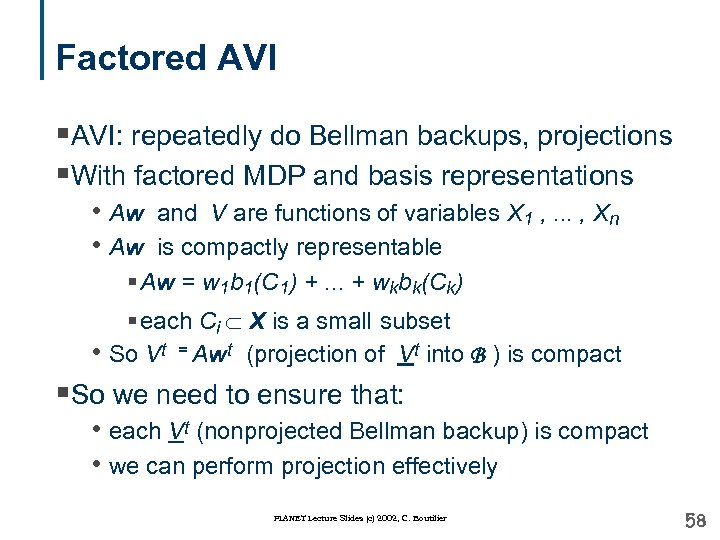Factored AVI §AVI: repeatedly do Bellman backups, projections §With factored MDP and basis representations • Aw and V are functions of variables X 1 , . . . , Xn • Aw is compactly representable § Aw = w 1 b 1(C 1) +. . . + wkbk(Ck) § each Ci X is a small subset • So Vt = Awt (projection of Vt into B ) is compact §So we need to ensure that: • each Vt (nonprojected Bellman backup) is compact • we can perform projection effectively PLANET Lecture Slides (c) 2002, C. Boutilier 58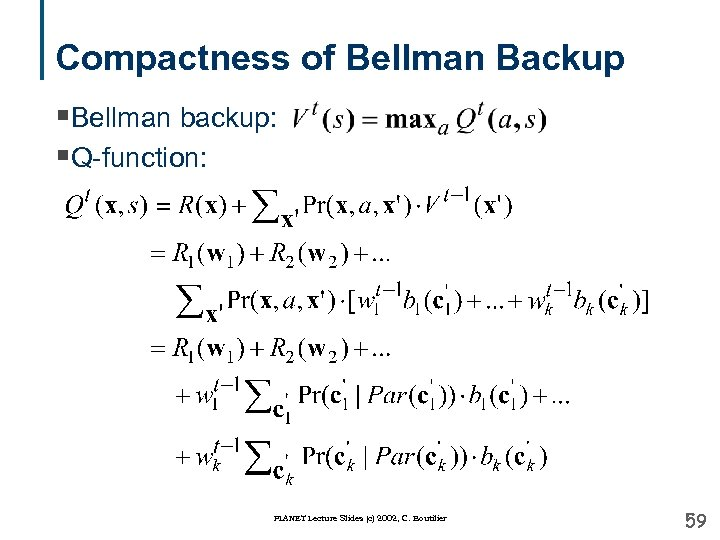Compactness of Bellman Backup §Bellman backup: §Q-function: PLANET Lecture Slides (c) 2002, C. Boutilier 59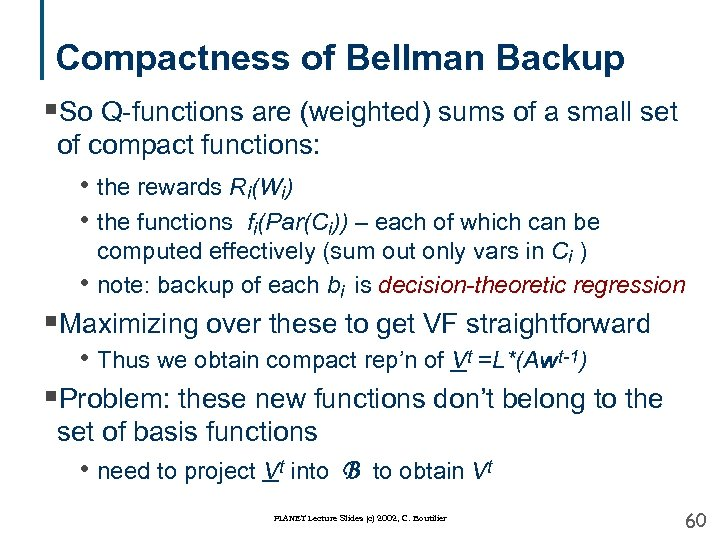Compactness of Bellman Backup §So Q-functions are (weighted) sums of a small set of compact functions: • the rewards Ri(Wi) • the functions fi(Par(Ci)) – each of which can be computed effectively (sum out only vars in Ci ) note: backup of each bi is decision-theoretic regression • §Maximizing over these to get VF straightforward • Thus we obtain compact rep’n of Vt =L*(Awt-1) §Problem: these new functions don’t belong to the set of basis functions • need to project Vt into B to obtain Vt PLANET Lecture Slides (c) 2002, C. Boutilier 60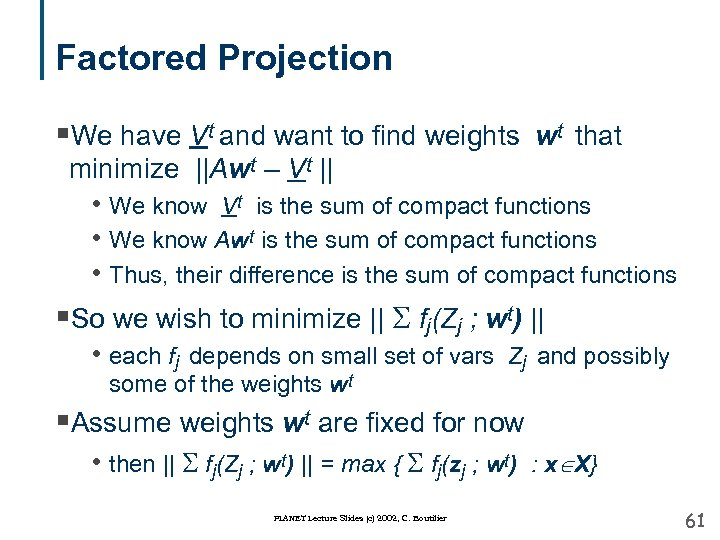Factored Projection §We have Vt and want to find weights wt that minimize ||Awt – Vt || • We know Vt is the sum of compact functions • We know Awt is the sum of compact functions • Thus, their difference is the sum of compact functions §So we wish to minimize || S fj(Zj ; wt) || • each fj depends on small set of vars Zj and possibly some of the weights wt §Assume weights wt are fixed for now • then || S fj(Zj ; wt) || = max { S fj(zj ; wt) : x X} PLANET Lecture Slides (c) 2002, C. Boutilier 61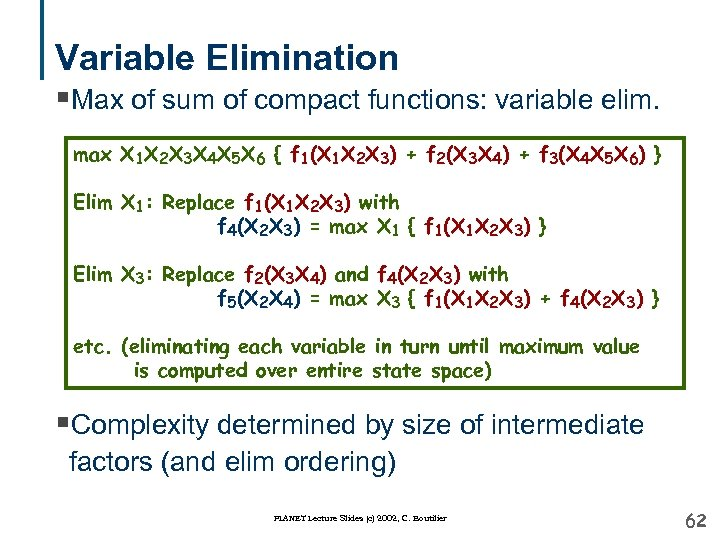Variable Elimination §Max of sum of compact functions: variable elim. max X 1 X 2 X 3 X 4 X 5 X 6 { f 1(X 1 X 2 X 3) + f 2(X 3 X 4) + f 3(X 4 X 5 X 6) } Elim X 1: Replace f 1(X 1 X 2 X 3) with f 4(X 2 X 3) = max X 1 { f 1(X 1 X 2 X 3) } Elim X 3: Replace f 2(X 3 X 4) and f 4(X 2 X 3) with f 5(X 2 X 4) = max X 3 { f 1(X 1 X 2 X 3) + f 4(X 2 X 3) } etc. (eliminating each variable in turn until maximum value is computed over entire state space) §Complexity determined by size of intermediate factors (and elim ordering) PLANET Lecture Slides (c) 2002, C. Boutilier 62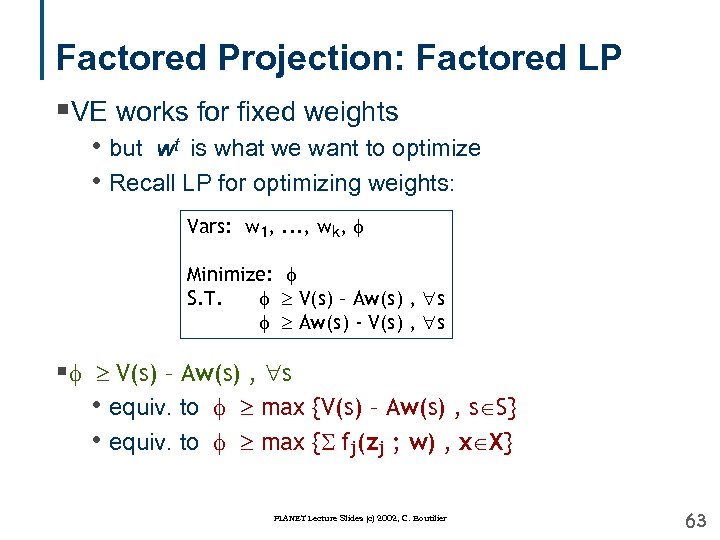Factored Projection: Factored LP §VE works for fixed weights • but wt is what we want to optimize • Recall LP for optimizing weights: Vars: w 1, . . . , wk, Minimize: S. T. V(s) – Aw(s) , s Aw(s) - V(s) , s § V(s) – Aw(s) , s • equiv. to max {V(s) – Aw(s) , s S} • equiv. to max {S fj(zj ; w) , x X} PLANET Lecture Slides (c) 2002, C. Boutilier 63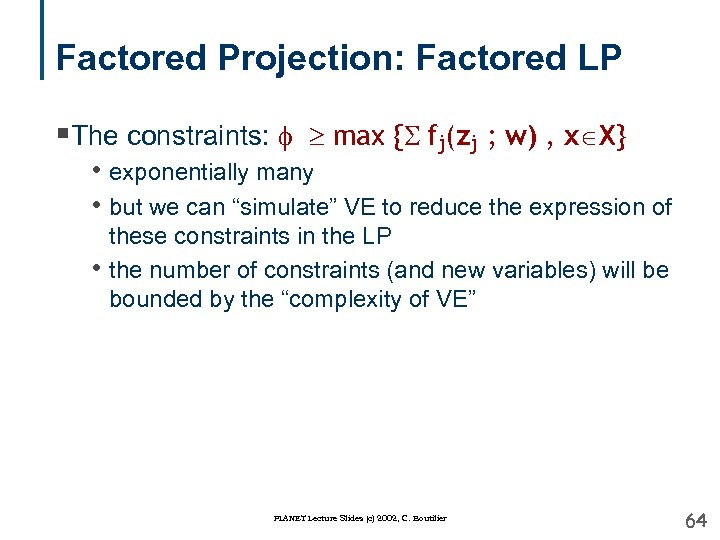Factored Projection: Factored LP §The constraints: max {S fj(zj ; w) , x X} • exponentially many • but we can “simulate” VE to reduce the expression of • these constraints in the LP the number of constraints (and new variables) will be bounded by the “complexity of VE” PLANET Lecture Slides (c) 2002, C. Boutilier 64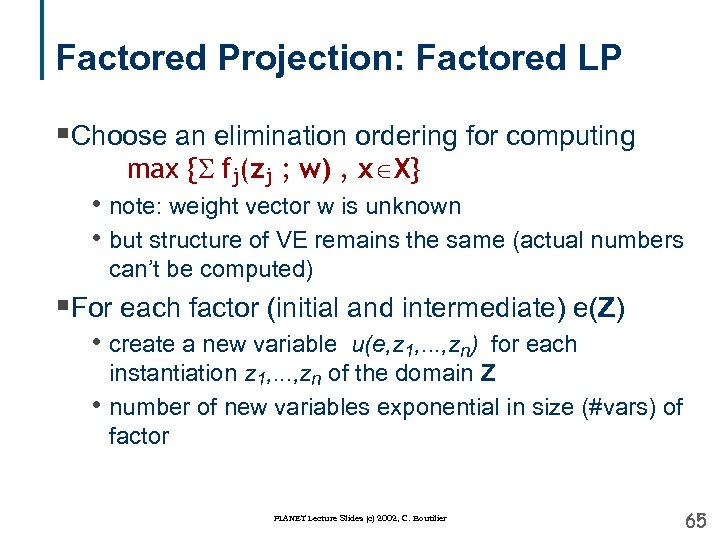Factored Projection: Factored LP §Choose an elimination ordering for computing max {S fj(zj ; w) , x X} • note: weight vector w is unknown • but structure of VE remains the same (actual numbers can’t be computed) §For each factor (initial and intermediate) e(Z) • create a new variable u(e, z 1, . . . , zn) for each • instantiation z 1, . . . , zn of the domain Z number of new variables exponential in size (#vars) of factor PLANET Lecture Slides (c) 2002, C. Boutilier 65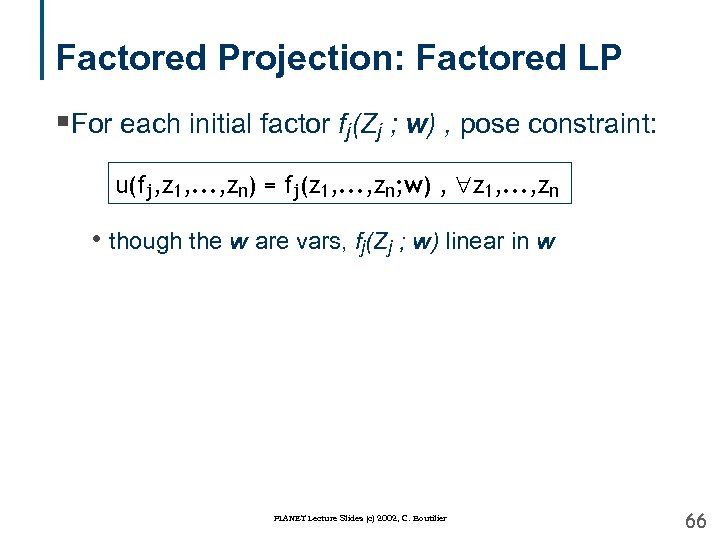Factored Projection: Factored LP §For each initial factor fj(Zj ; w) , pose constraint: u(fj, z 1, . . . , zn) = fj(z 1, . . . , zn; w) , z 1, . . . , zn • though the w are vars, fj(Zj ; w) linear in w PLANET Lecture Slides (c) 2002, C. Boutilier 66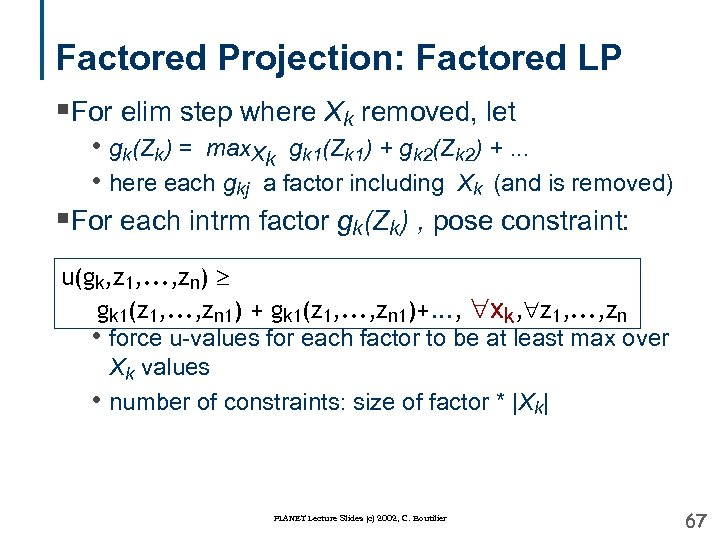Factored Projection: Factored LP §For elim step where Xk removed, let • gk(Zk) = max. Xk gk 1(Zk 1) + gk 2(Zk 2) +. . . • here each gkj a factor including Xk (and is removed) §For each intrm factor gk(Zk) , pose constraint: u(gk, z 1, . . . , zn) gk 1(z 1, . . . , zn 1) + gk 1(z 1, . . . , zn 1)+. . . , xk, z 1, . . . , zn • force u-values for each factor to be at least max over Xk values • number of constraints: size of factor * |Xk| PLANET Lecture Slides (c) 2002, C. Boutilier 67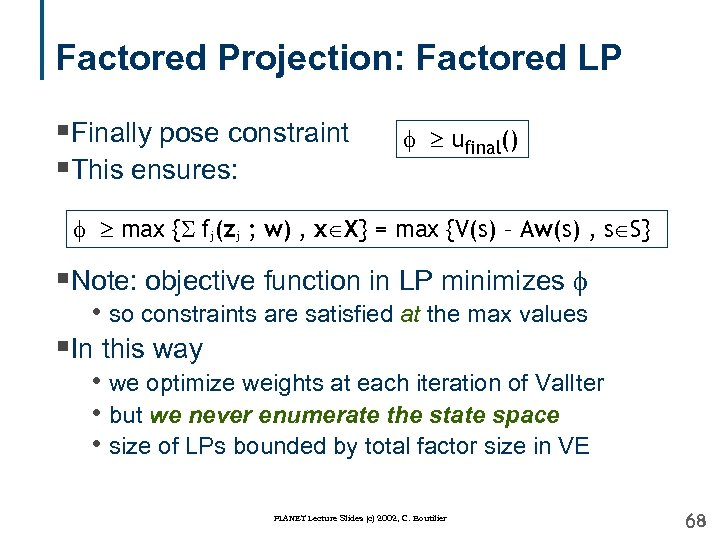Factored Projection: Factored LP §Finally pose constraint §This ensures: ufinal() max {S fj(zj ; w) , x X} = max {V(s) – Aw(s) , s S} §Note: objective function in LP minimizes • so constraints are satisfied at the max values §In this way • we optimize weights at each iteration of Val. Iter • but we never enumerate the state space • size of LPs bounded by total factor size in VE PLANET Lecture Slides (c) 2002, C. Boutilier 68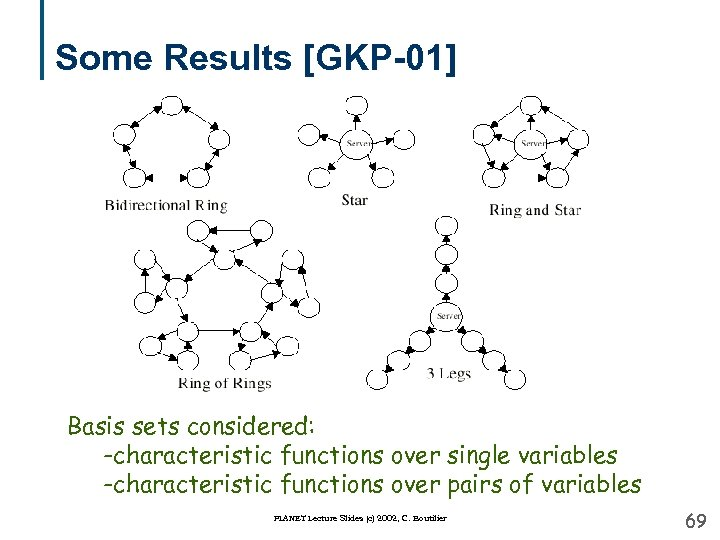Some Results [GKP-01] Basis sets considered: -characteristic functions over single variables -characteristic functions over pairs of variables PLANET Lecture Slides (c) 2002, C. Boutilier 69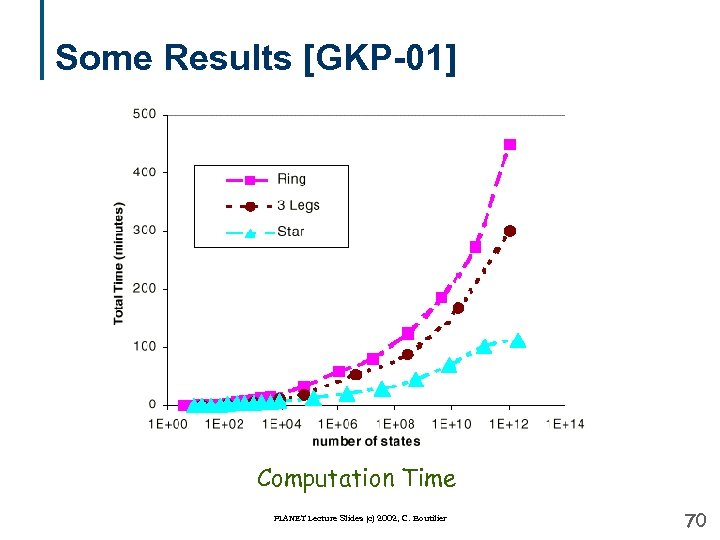Some Results [GKP-01] Computation Time PLANET Lecture Slides (c) 2002, C. Boutilier 70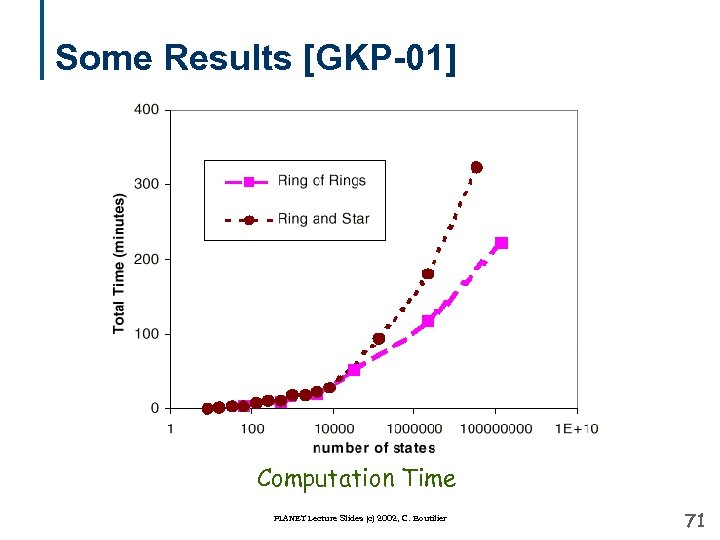Some Results [GKP-01] Computation Time PLANET Lecture Slides (c) 2002, C. Boutilier 71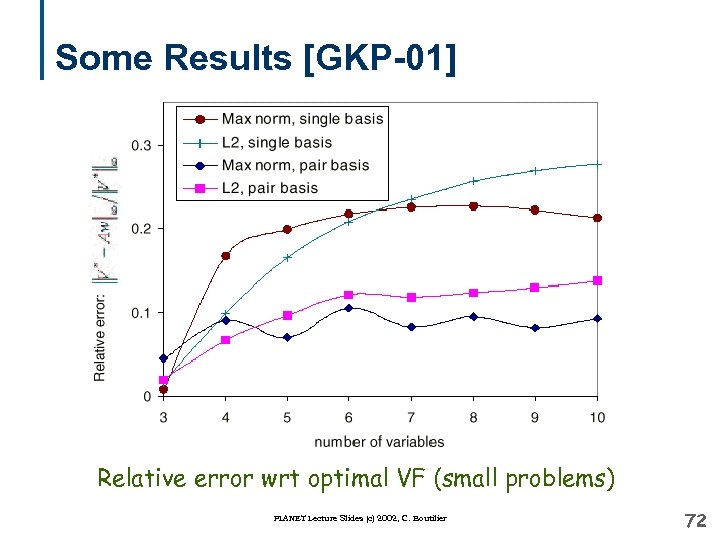Some Results [GKP-01] Relative error wrt optimal VF (small problems) PLANET Lecture Slides (c) 2002, C. Boutilier 72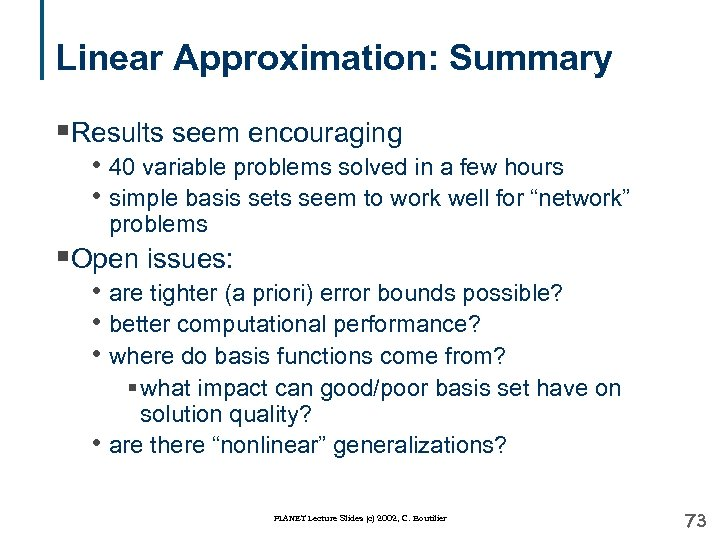Linear Approximation: Summary §Results seem encouraging • 40 variable problems solved in a few hours • simple basis sets seem to work well for “network” problems §Open issues: • are tighter (a priori) error bounds possible? • better computational performance? • where do basis functions come from? § what impact can good/poor basis set have on solution quality? • are there “nonlinear” generalizations? PLANET Lecture Slides (c) 2002, C. Boutilier 73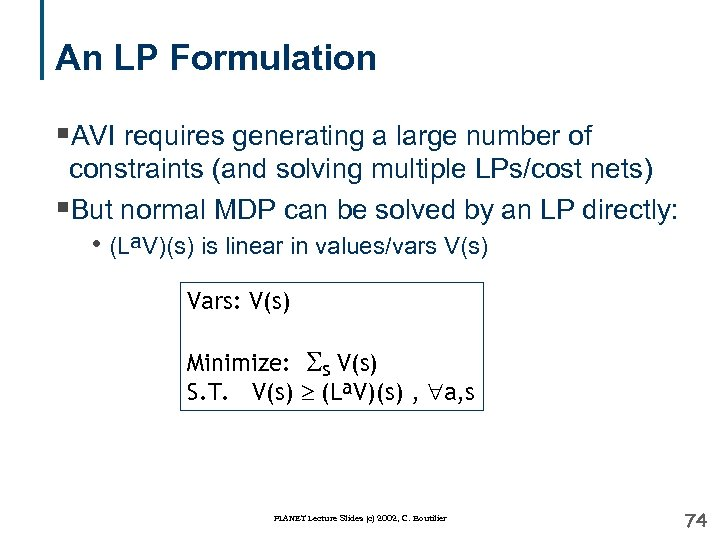An LP Formulation §AVI requires generating a large number of constraints (and solving multiple LPs/cost nets) §But normal MDP can be solved by an LP directly: • (La. V)(s) is linear in values/vars V(s) Vars: V(s) Minimize: Ss V(s) S. T. V(s) (La. V)(s) , a, s PLANET Lecture Slides (c) 2002, C. Boutilier 74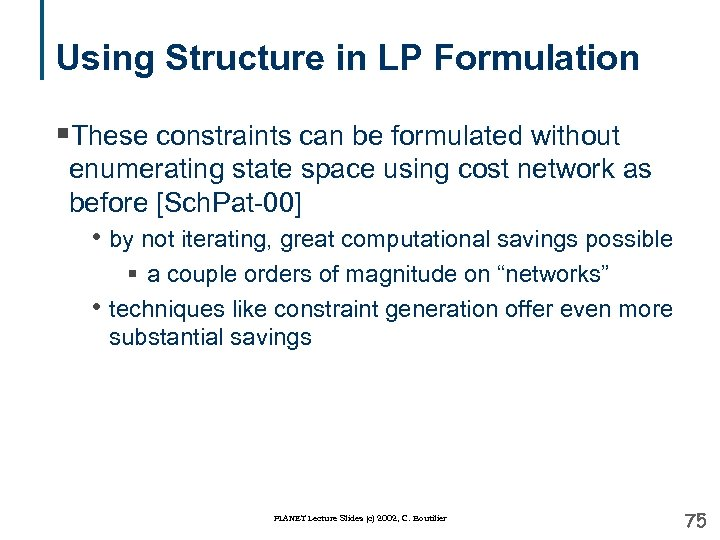Using Structure in LP Formulation §These constraints can be formulated without enumerating state space using cost network as before [Sch. Pat-00] • by not iterating, great computational savings possible § a couple orders of magnitude on “networks” • techniques like constraint generation offer even more substantial savings PLANET Lecture Slides (c) 2002, C. Boutilier 75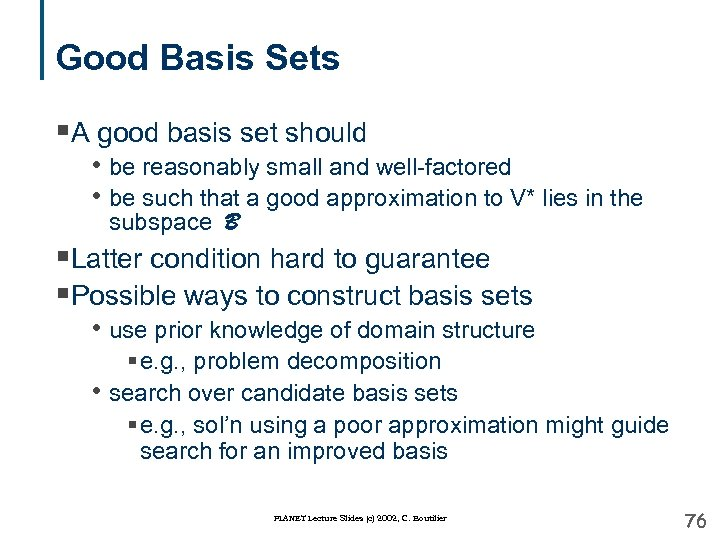Good Basis Sets §A good basis set should • be reasonably small and well-factored • be such that a good approximation to V* lies in the subspace B §Latter condition hard to guarantee §Possible ways to construct basis sets • use prior knowledge of domain structure § e. g. , problem decomposition • search over candidate basis sets § e. g. , sol’n using a poor approximation might guide search for an improved basis PLANET Lecture Slides (c) 2002, C. Boutilier 76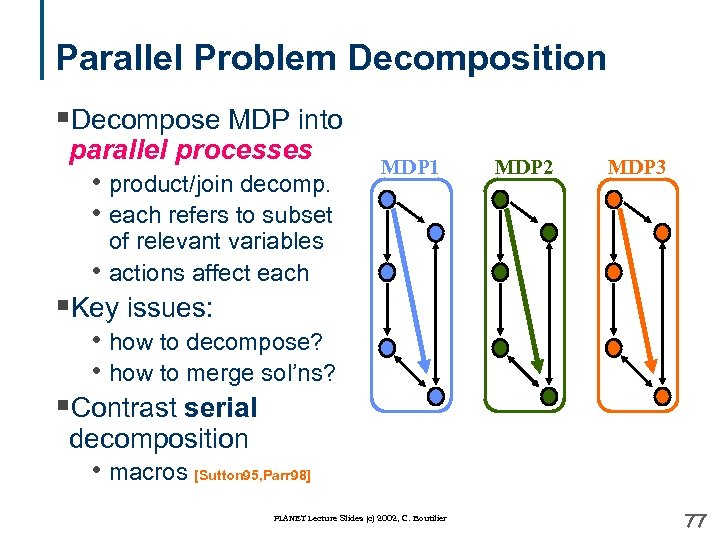Parallel Problem Decomposition §Decompose MDP into parallel processes • product/join decomp. • each refers to subset MDP 1 MDP 2 MDP 3 of relevant variables actions affect each • §Key issues: • how to decompose? • how to merge sol’ns? §Contrast serial decomposition • macros [Sutton 95, Parr 98] PLANET Lecture Slides (c) 2002, C. Boutilier 77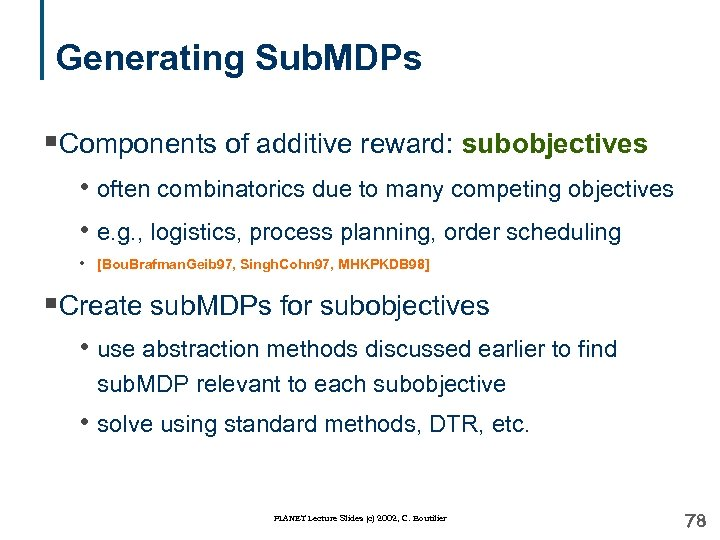Generating Sub. MDPs §Components of additive reward: subobjectives • often combinatorics due to many competing objectives • e. g. , logistics, process planning, order scheduling • [Bou. Brafman. Geib 97, Singh. Cohn 97, MHKPKDB 98] §Create sub. MDPs for subobjectives • use abstraction methods discussed earlier to find sub. MDP relevant to each subobjective • solve using standard methods, DTR, etc. PLANET Lecture Slides (c) 2002, C. Boutilier 78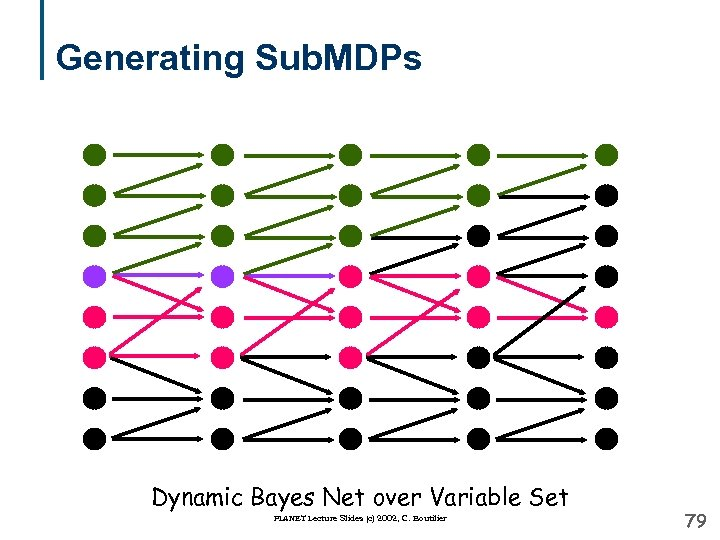Generating Sub. MDPs Dynamic Bayes Net over Variable Set PLANET Lecture Slides (c) 2002, C. Boutilier 79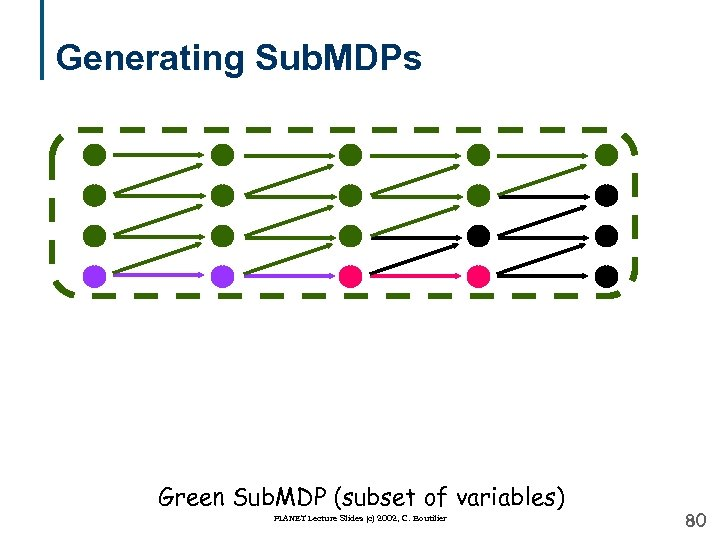Generating Sub. MDPs Green Sub. MDP (subset of variables) PLANET Lecture Slides (c) 2002, C. Boutilier 80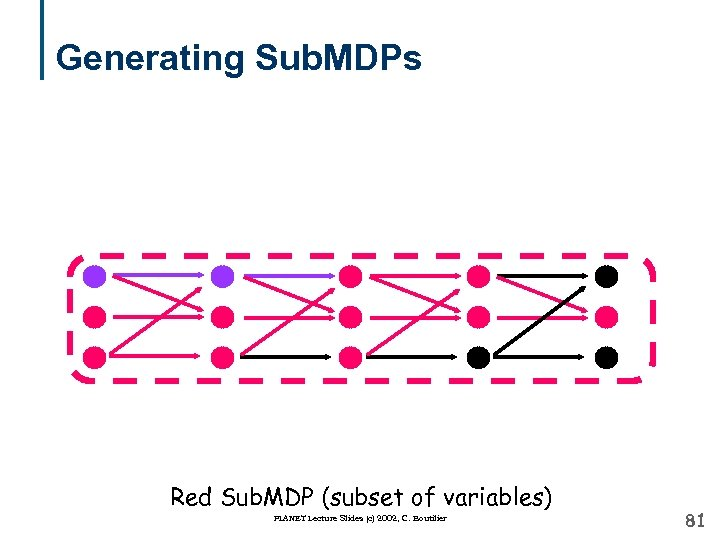Generating Sub. MDPs Red Sub. MDP (subset of variables) PLANET Lecture Slides (c) 2002, C. Boutilier 81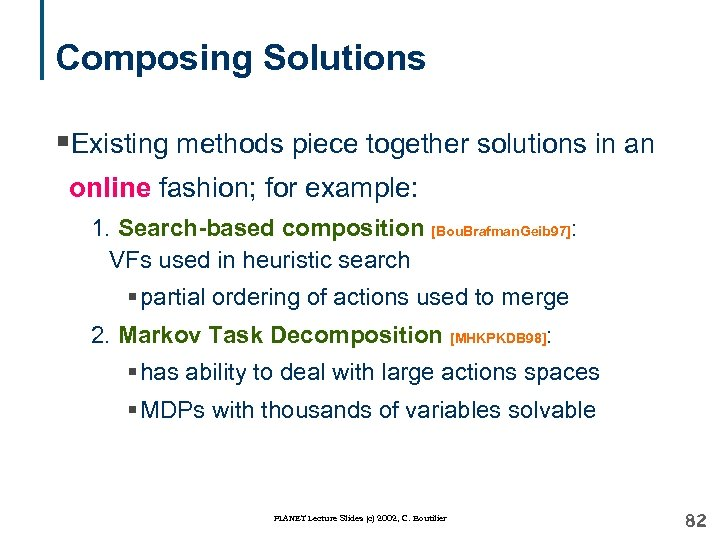Composing Solutions §Existing methods piece together solutions in an online fashion; for example: 1. Search-based composition [Bou. Brafman. Geib 97]: VFs used in heuristic search § partial ordering of actions used to merge 2. Markov Task Decomposition [MHKPKDB 98]: § has ability to deal with large actions spaces § MDPs with thousands of variables solvable PLANET Lecture Slides (c) 2002, C. Boutilier 82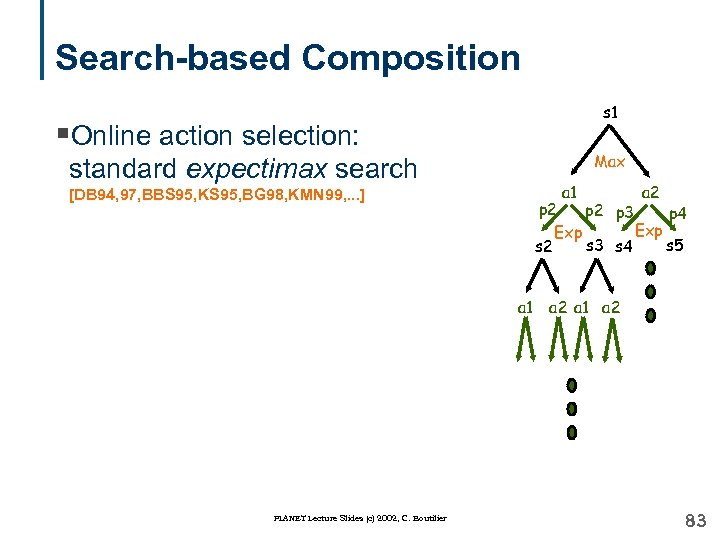Search-based Composition s 1 §Online action selection: Max standard expectimax search a 1 [DB 94, 97, BBS 95, KS 95, BG 98, KMN 99, . . . ] p 2 p 3 p 4 Exp s 3 s 4 s 5 s 2 a 1 PLANET Lecture Slides (c) 2002, C. Boutilier a 2 a 1 a 2 83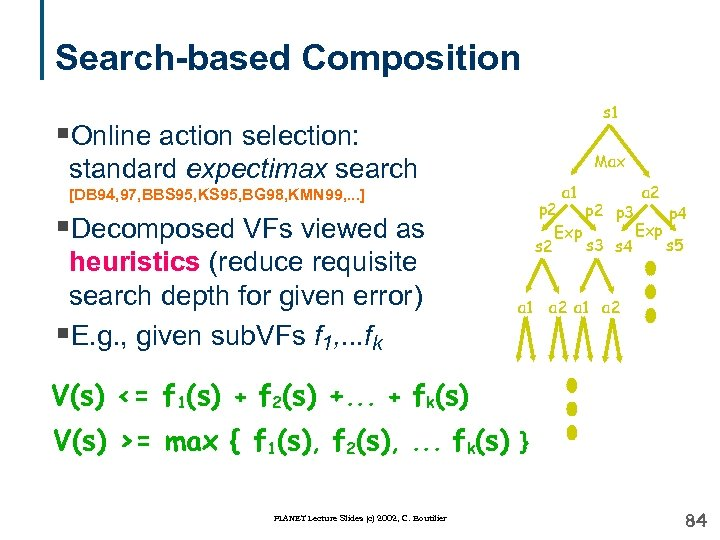Search-based Composition s 1 §Online action selection: Max standard expectimax search a 1 [DB 94, 97, BBS 95, KS 95, BG 98, KMN 99, . . . ] p 2 p 3 p 4 Exp s 3 s 4 s 5 s 2 §Decomposed VFs viewed as heuristics (reduce requisite search depth for given error) §E. g. , given sub. VFs f 1, . . . fk a 2 a 1 a 2 V(s) <= f 1(s) + f 2(s) +. . . + fk(s) V(s) >= max { f 1(s), f 2(s), . . . fk(s) } PLANET Lecture Slides (c) 2002, C. Boutilier 84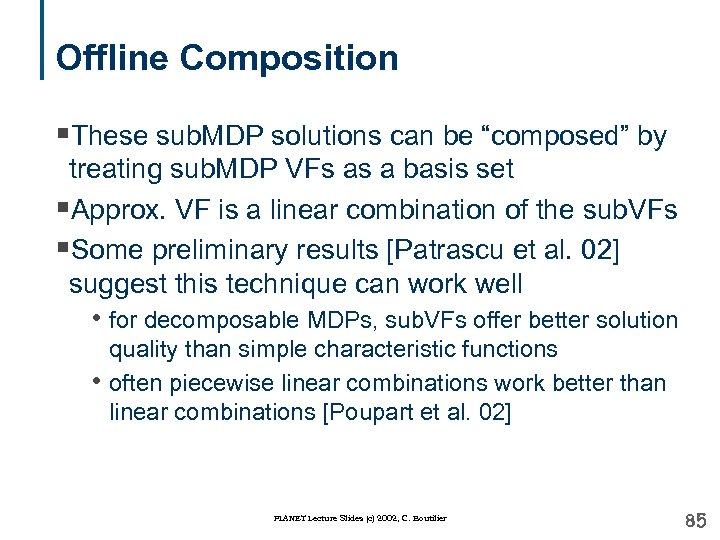Offline Composition §These sub. MDP solutions can be “composed” by treating sub. MDP VFs as a basis set §Approx. VF is a linear combination of the sub. VFs §Some preliminary results [Patrascu et al. 02] suggest this technique can work well • for decomposable MDPs, sub. VFs offer better solution • quality than simple characteristic functions often piecewise linear combinations work better than linear combinations [Poupart et al. 02] PLANET Lecture Slides (c) 2002, C. Boutilier 85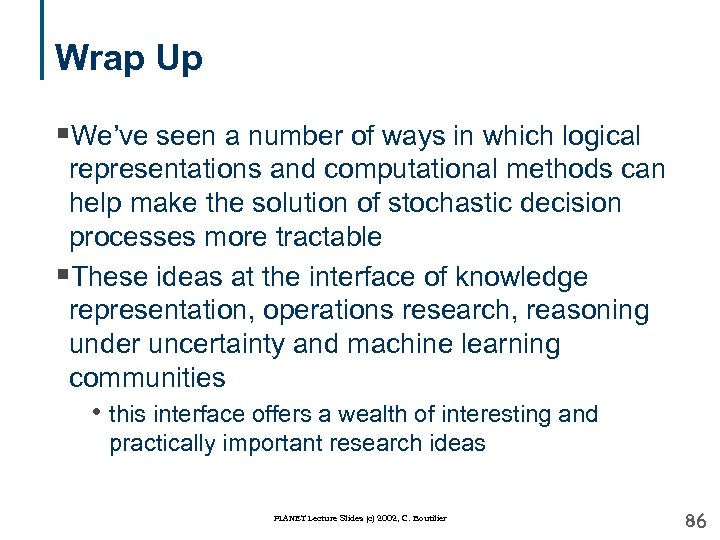Wrap Up §We’ve seen a number of ways in which logical representations and computational methods can help make the solution of stochastic decision processes more tractable §These ideas at the interface of knowledge representation, operations research, reasoning under uncertainty and machine learning communities • this interface offers a wealth of interesting and practically important research ideas PLANET Lecture Slides (c) 2002, C. Boutilier 86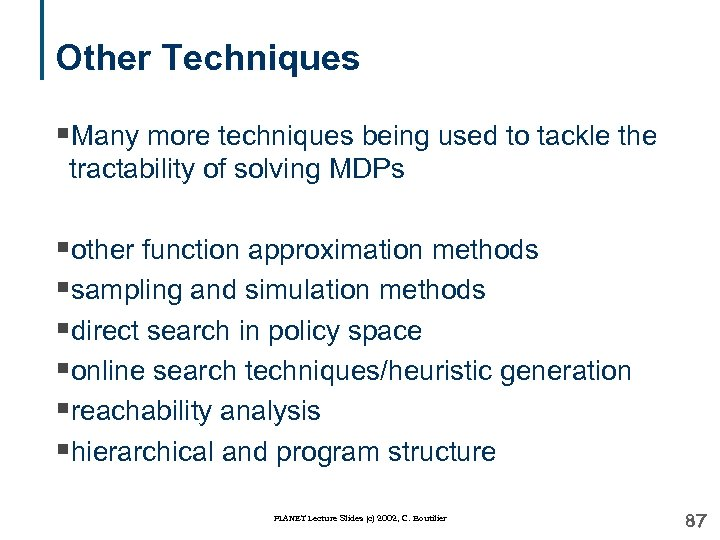Other Techniques §Many more techniques being used to tackle the tractability of solving MDPs §other function approximation methods §sampling and simulation methods §direct search in policy space §online search techniques/heuristic generation §reachability analysis §hierarchical and program structure PLANET Lecture Slides (c) 2002, C. Boutilier 87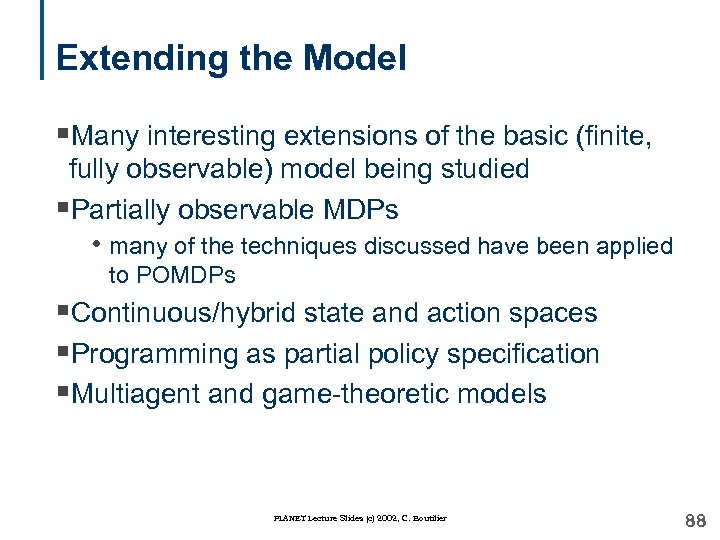Extending the Model §Many interesting extensions of the basic (finite, fully observable) model being studied §Partially observable MDPs • many of the techniques discussed have been applied to POMDPs §Continuous/hybrid state and action spaces §Programming as partial policy specification §Multiagent and game-theoretic models PLANET Lecture Slides (c) 2002, C. Boutilier 88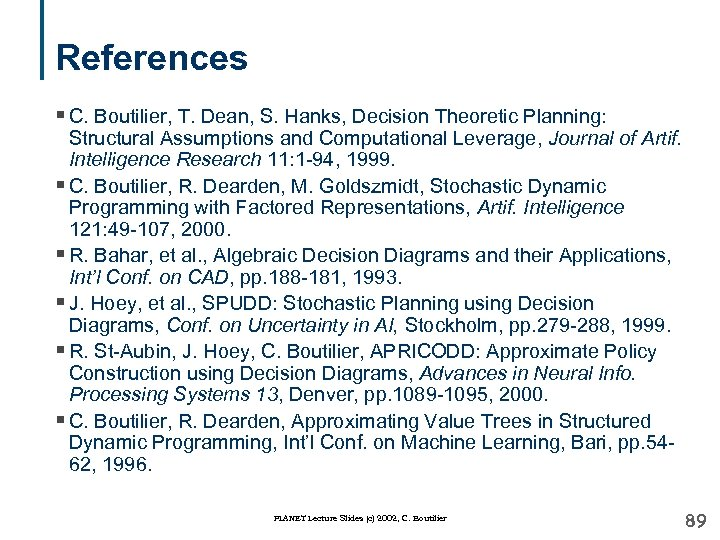References § C. Boutilier, T. Dean, S. Hanks, Decision Theoretic Planning: Structural Assumptions and Computational Leverage, Journal of Artif. Intelligence Research 11: 1 -94, 1999. § C. Boutilier, R. Dearden, M. Goldszmidt, Stochastic Dynamic Programming with Factored Representations, Artif. Intelligence 121: 49 -107, 2000. § R. Bahar, et al. , Algebraic Decision Diagrams and their Applications, Int’l Conf. on CAD, pp. 188 -181, 1993. § J. Hoey, et al. , SPUDD: Stochastic Planning using Decision Diagrams, Conf. on Uncertainty in AI, Stockholm, pp. 279 -288, 1999. § R. St-Aubin, J. Hoey, C. Boutilier, APRICODD: Approximate Policy Construction using Decision Diagrams, Advances in Neural Info. Processing Systems 13, Denver, pp. 1089 -1095, 2000. § C. Boutilier, R. Dearden, Approximating Value Trees in Structured Dynamic Programming, Int’l Conf. on Machine Learning, Bari, pp. 5462, 1996. PLANET Lecture Slides (c) 2002, C. Boutilier 89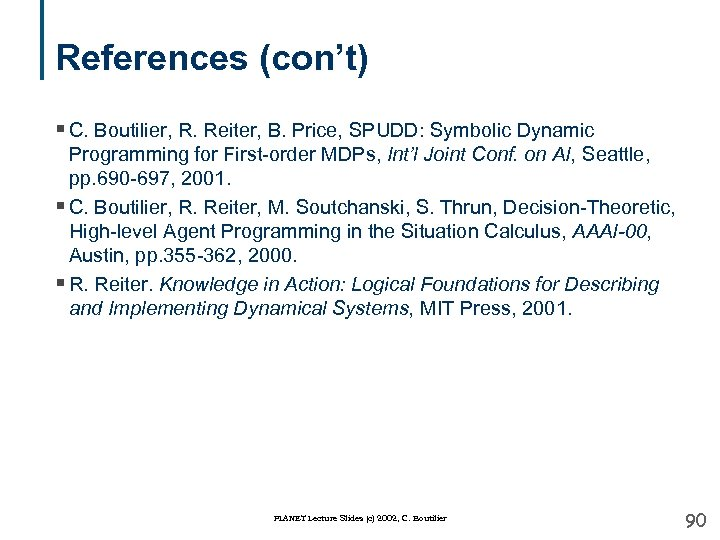References (con’t) § C. Boutilier, R. Reiter, B. Price, SPUDD: Symbolic Dynamic Programming for First-order MDPs, Int’l Joint Conf. on AI, Seattle, pp. 690 -697, 2001. § C. Boutilier, R. Reiter, M. Soutchanski, S. Thrun, Decision-Theoretic, High-level Agent Programming in the Situation Calculus, AAAI-00, Austin, pp. 355 -362, 2000. § R. Reiter. Knowledge in Action: Logical Foundations for Describing and Implementing Dynamical Systems, MIT Press, 2001. PLANET Lecture Slides (c) 2002, C. Boutilier 90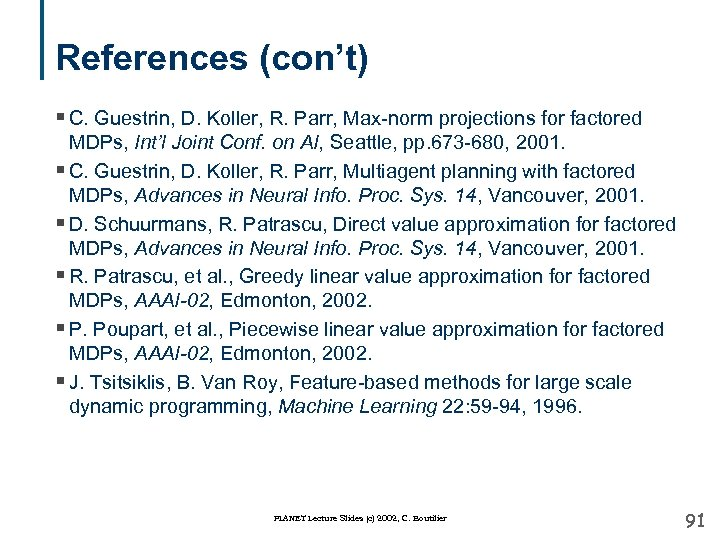References (con’t) § C. Guestrin, D. Koller, R. Parr, Max-norm projections for factored MDPs, Int’l Joint Conf. on AI, Seattle, pp. 673 -680, 2001. § C. Guestrin, D. Koller, R. Parr, Multiagent planning with factored MDPs, Advances in Neural Info. Proc. Sys. 14, Vancouver, 2001. § D. Schuurmans, R. Patrascu, Direct value approximation for factored MDPs, Advances in Neural Info. Proc. Sys. 14, Vancouver, 2001. § R. Patrascu, et al. , Greedy linear value approximation for factored MDPs, AAAI-02, Edmonton, 2002. § P. Poupart, et al. , Piecewise linear value approximation for factored MDPs, AAAI-02, Edmonton, 2002. § J. Tsitsiklis, B. Van Roy, Feature-based methods for large scale dynamic programming, Machine Learning 22: 59 -94, 1996. PLANET Lecture Slides (c) 2002, C. Boutilier 91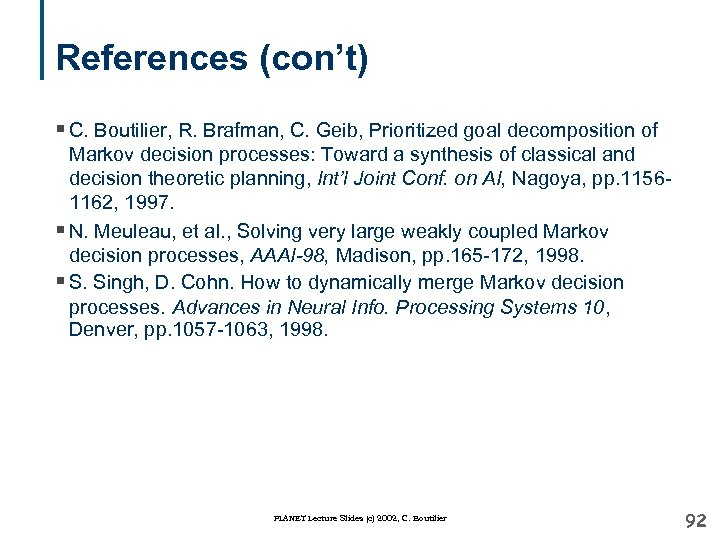References (con’t) § C. Boutilier, R. Brafman, C. Geib, Prioritized goal decomposition of Markov decision processes: Toward a synthesis of classical and decision theoretic planning, Int’l Joint Conf. on AI, Nagoya, pp. 11561162, 1997. § N. Meuleau, et al. , Solving very large weakly coupled Markov decision processes, AAAI-98, Madison, pp. 165 -172, 1998. § S. Singh, D. Cohn. How to dynamically merge Markov decision processes. Advances in Neural Info. Processing Systems 10, Denver, pp. 1057 -1063, 1998. PLANET Lecture Slides (c) 2002, C. Boutilier 92